# Concise Selina Solutions for Class 9 Maths Chapter 27- Graphical Solution

Selina Solutions are useful for students as it helps them in scoring high marks in the examination. The Selina Solutions given in this page contains detailed step-by-step explanations of all the problems that come under Chapter 27, Graphical Solution, of the Class 9 Selina Textbook. This chapter deals with the Solution of Simultaneous Linear Equations, Graphically.

These solutions are prepared by subject matter experts at BYJU’S, describing the complete method of solving problems. By understanding the concepts used in Selina Solutions for Class 9 Maths, students will be able to clear all their doubts related to “Graphical Solution”.

### Download PDF of Selina Solutions for Class 9 Maths Chapter 27:-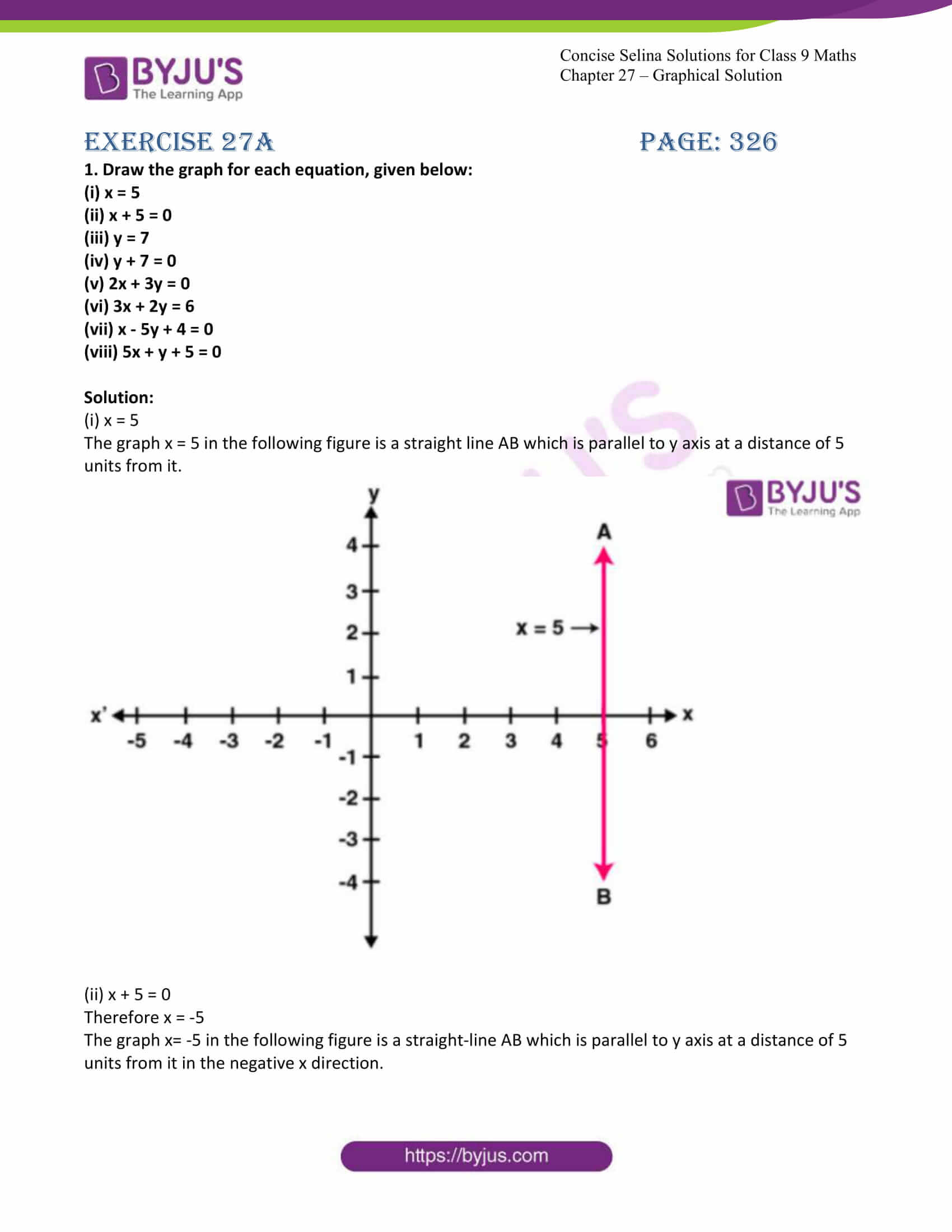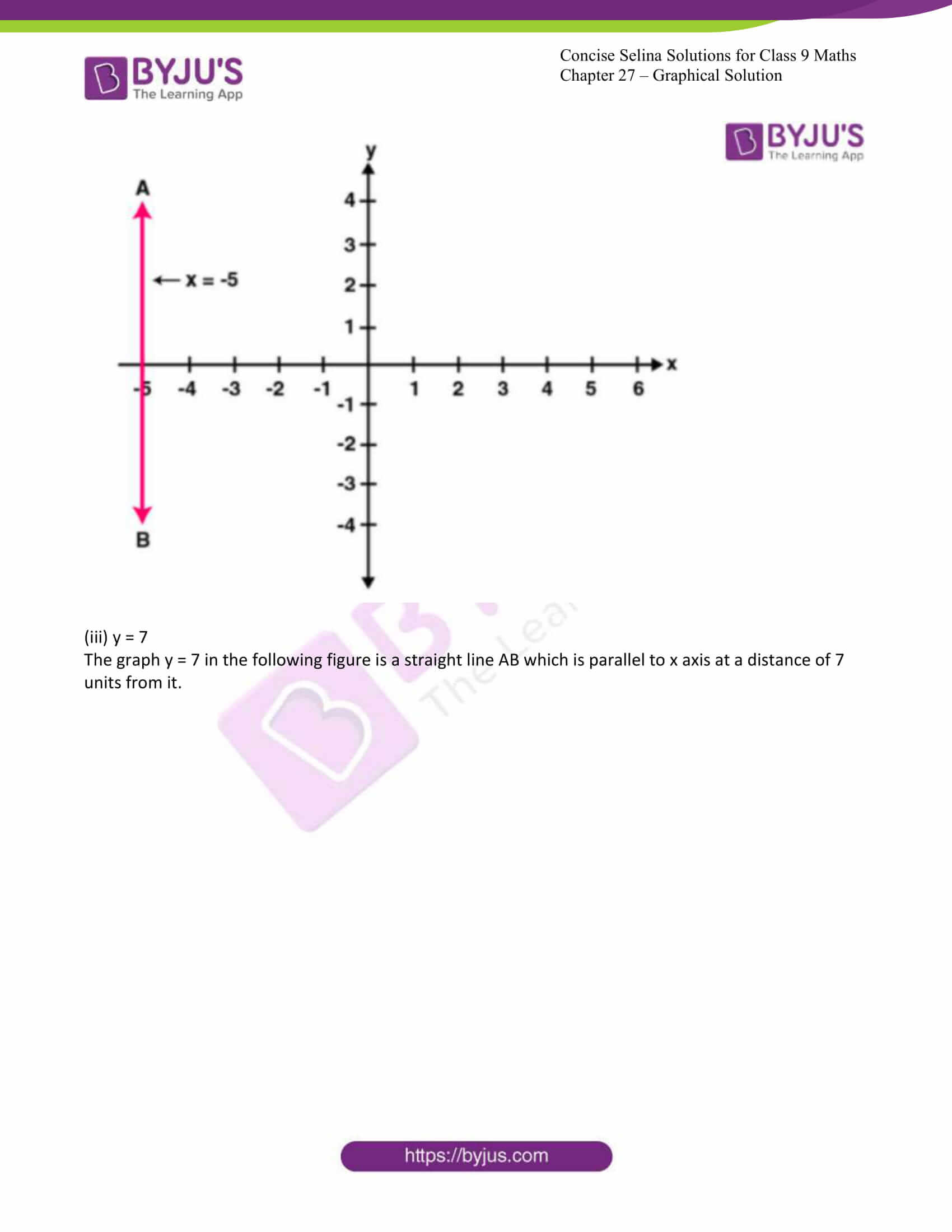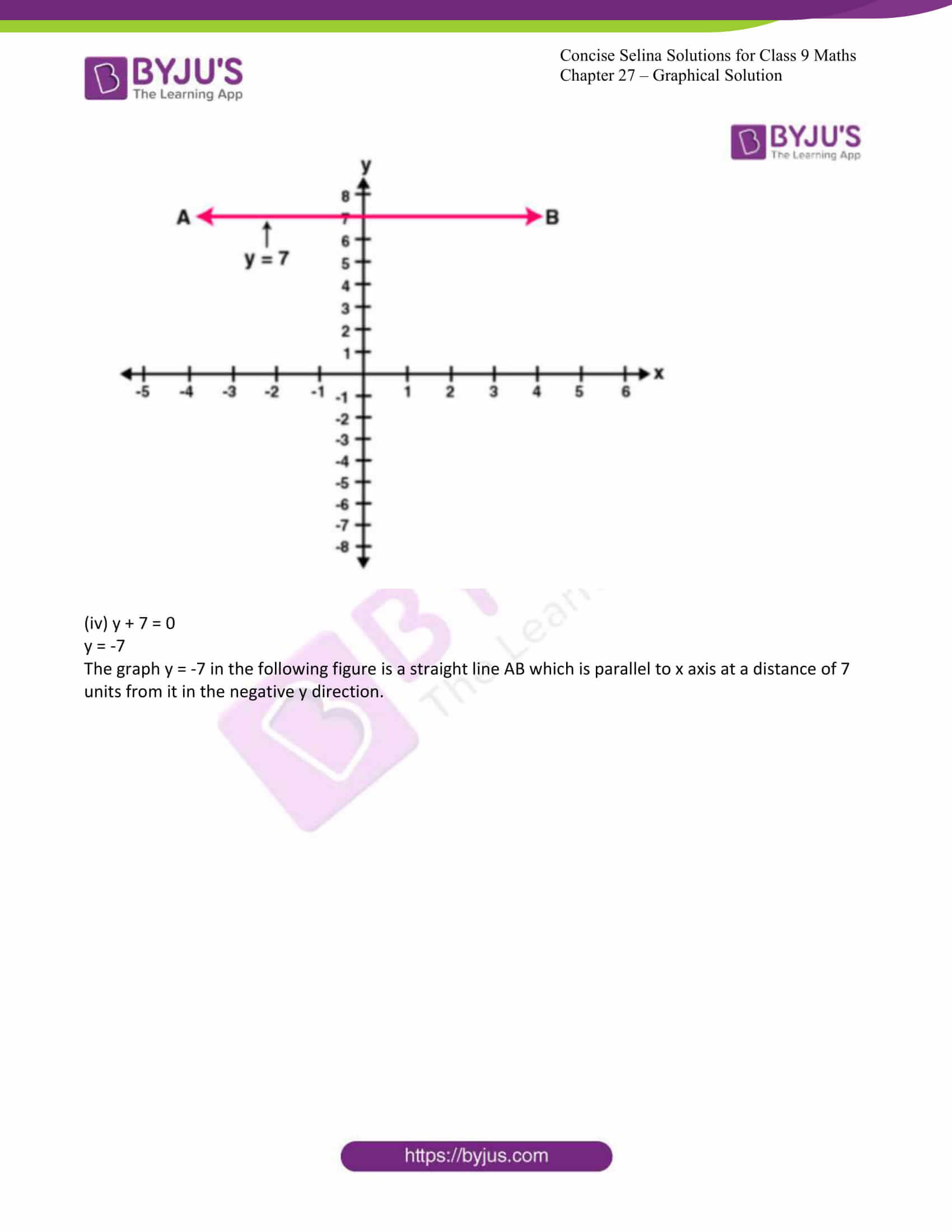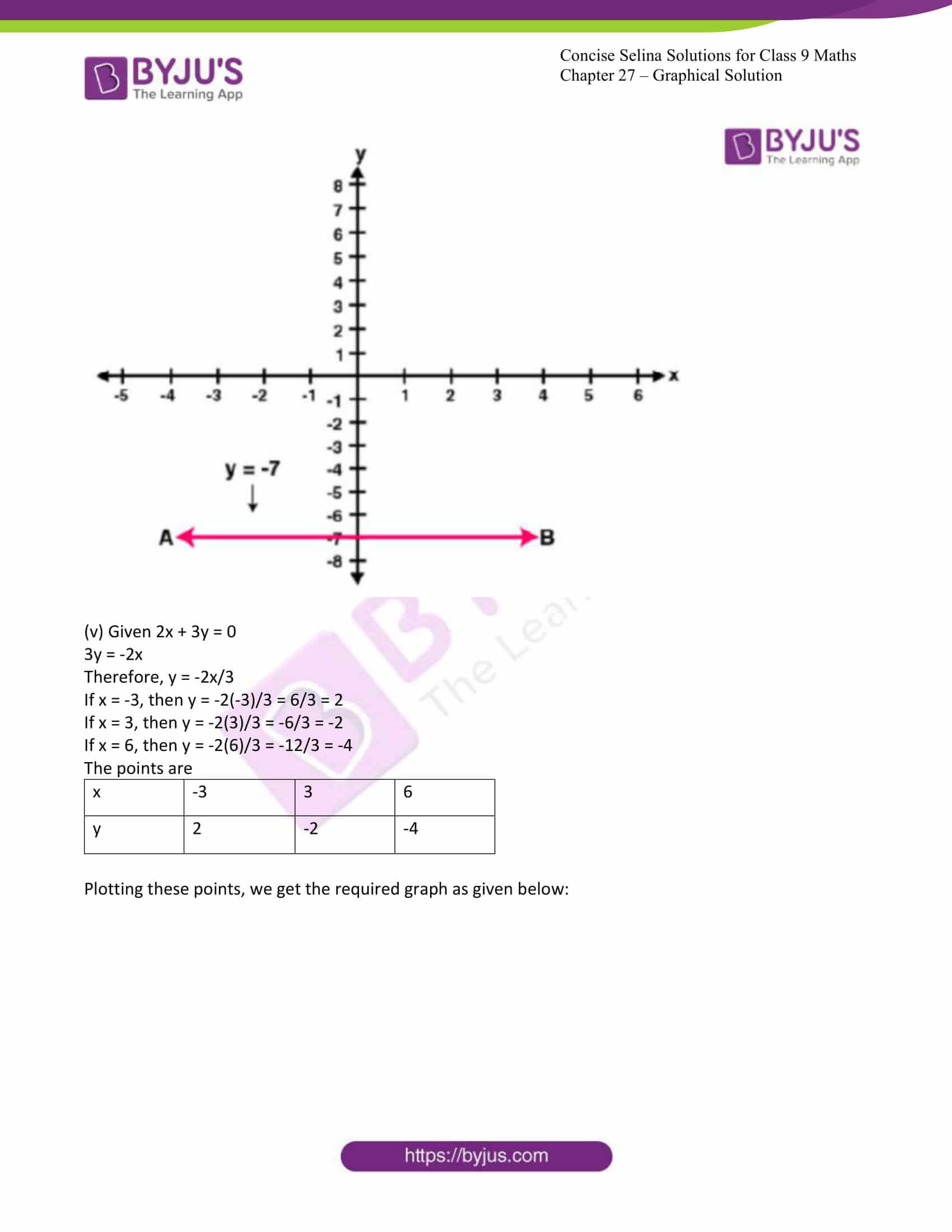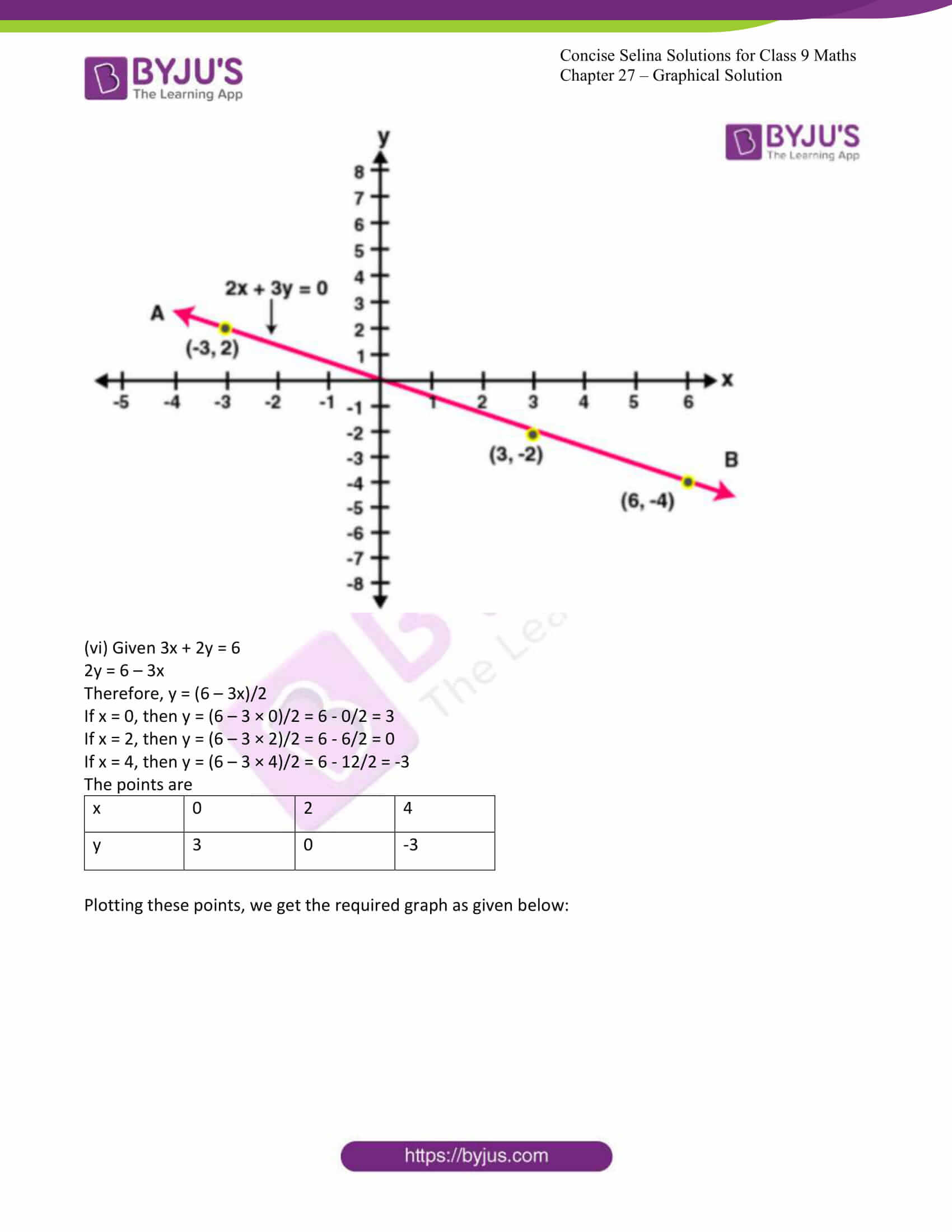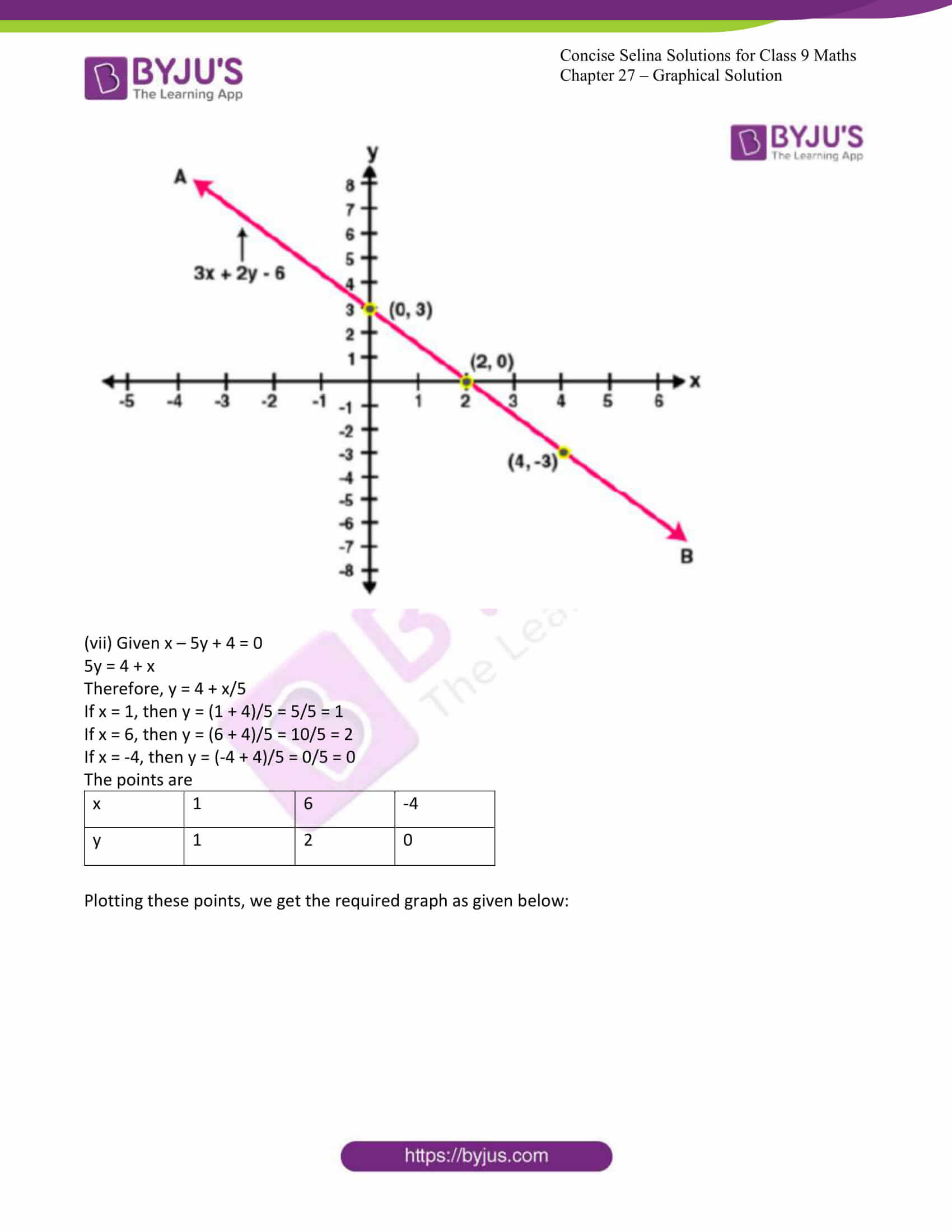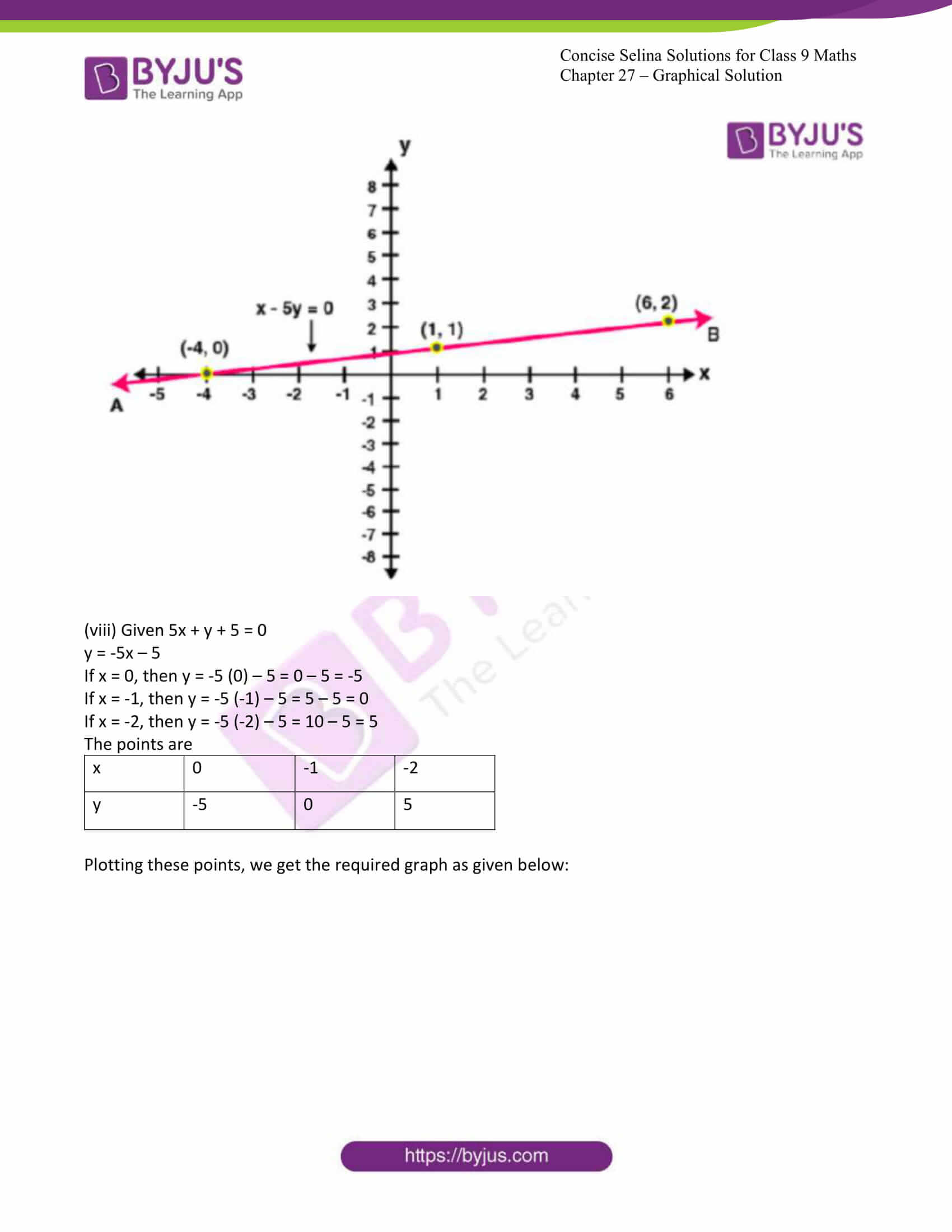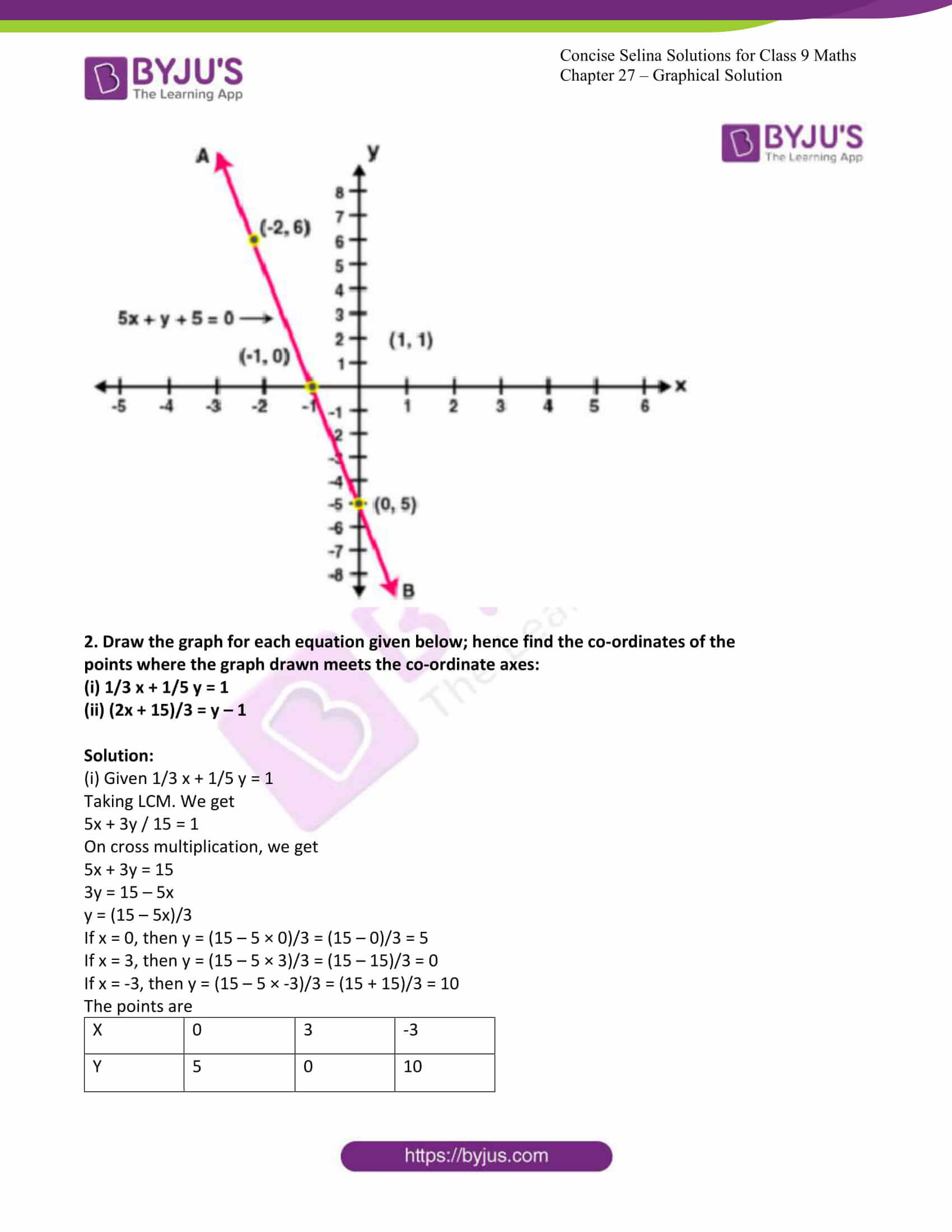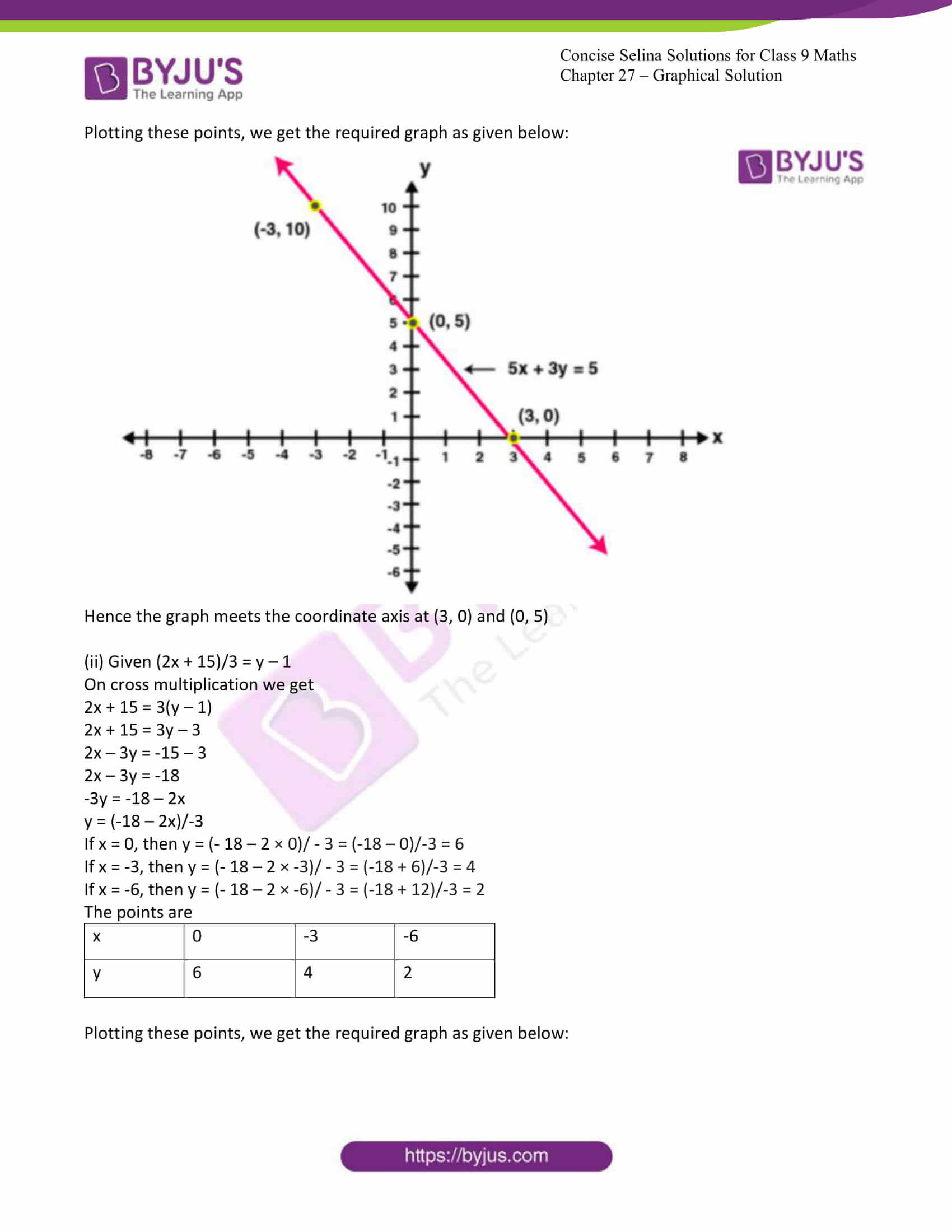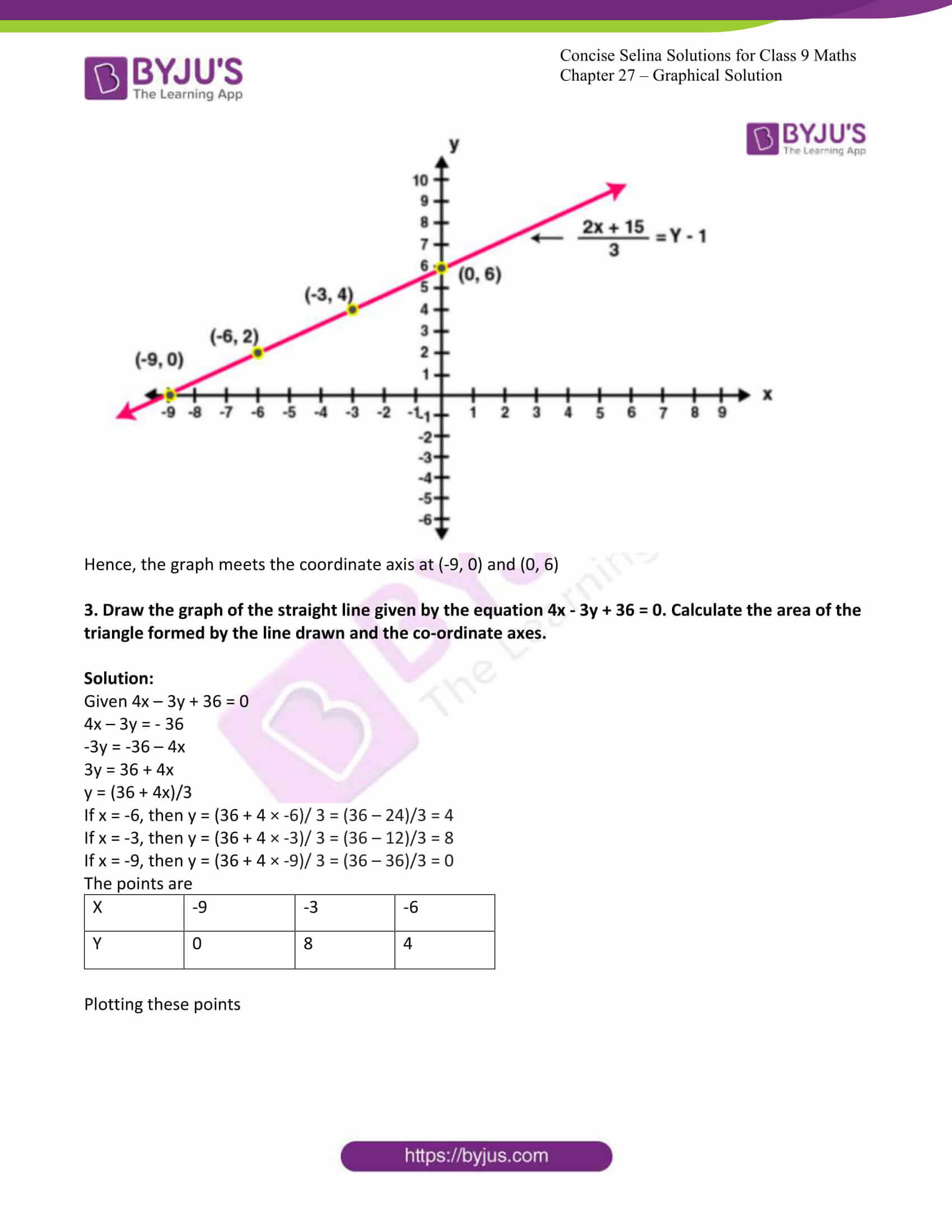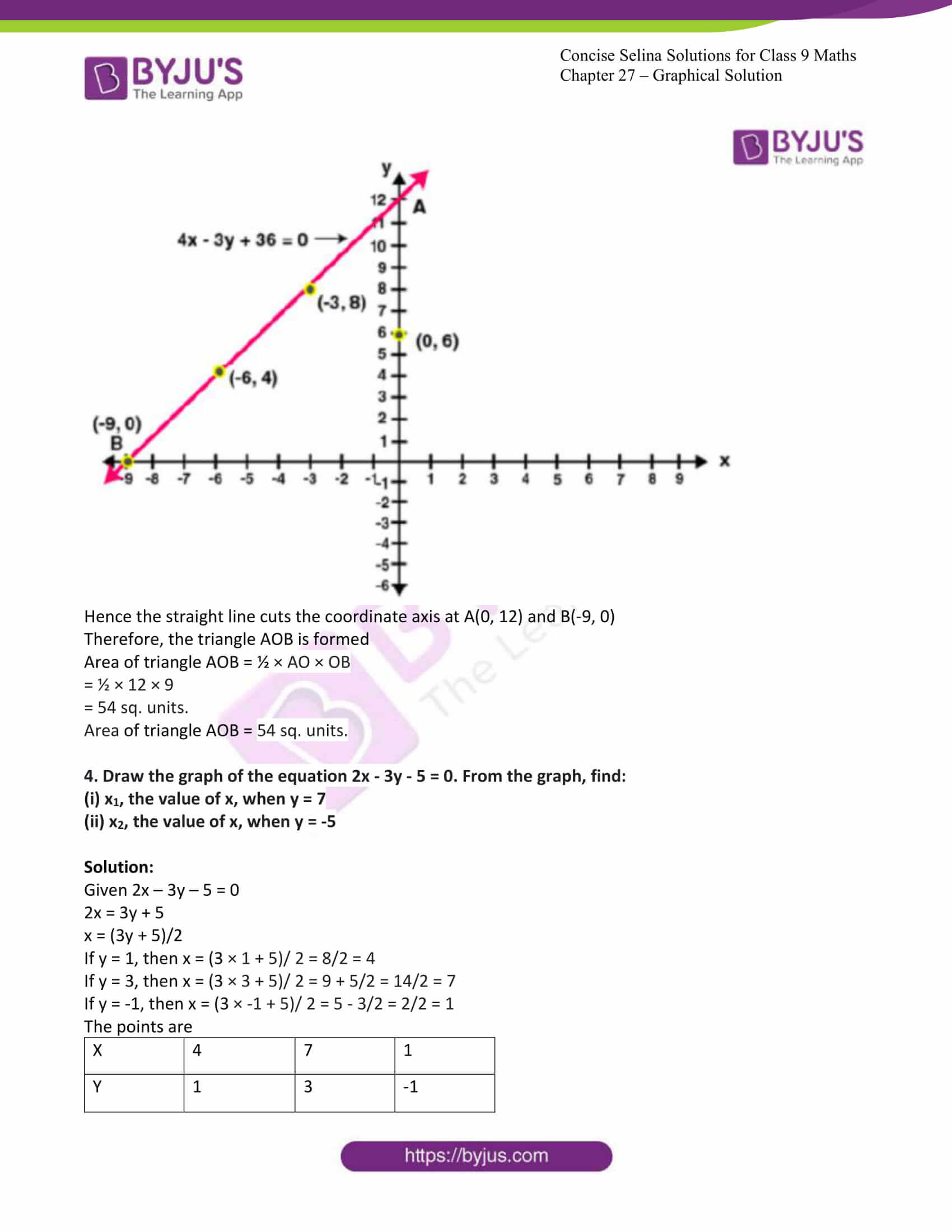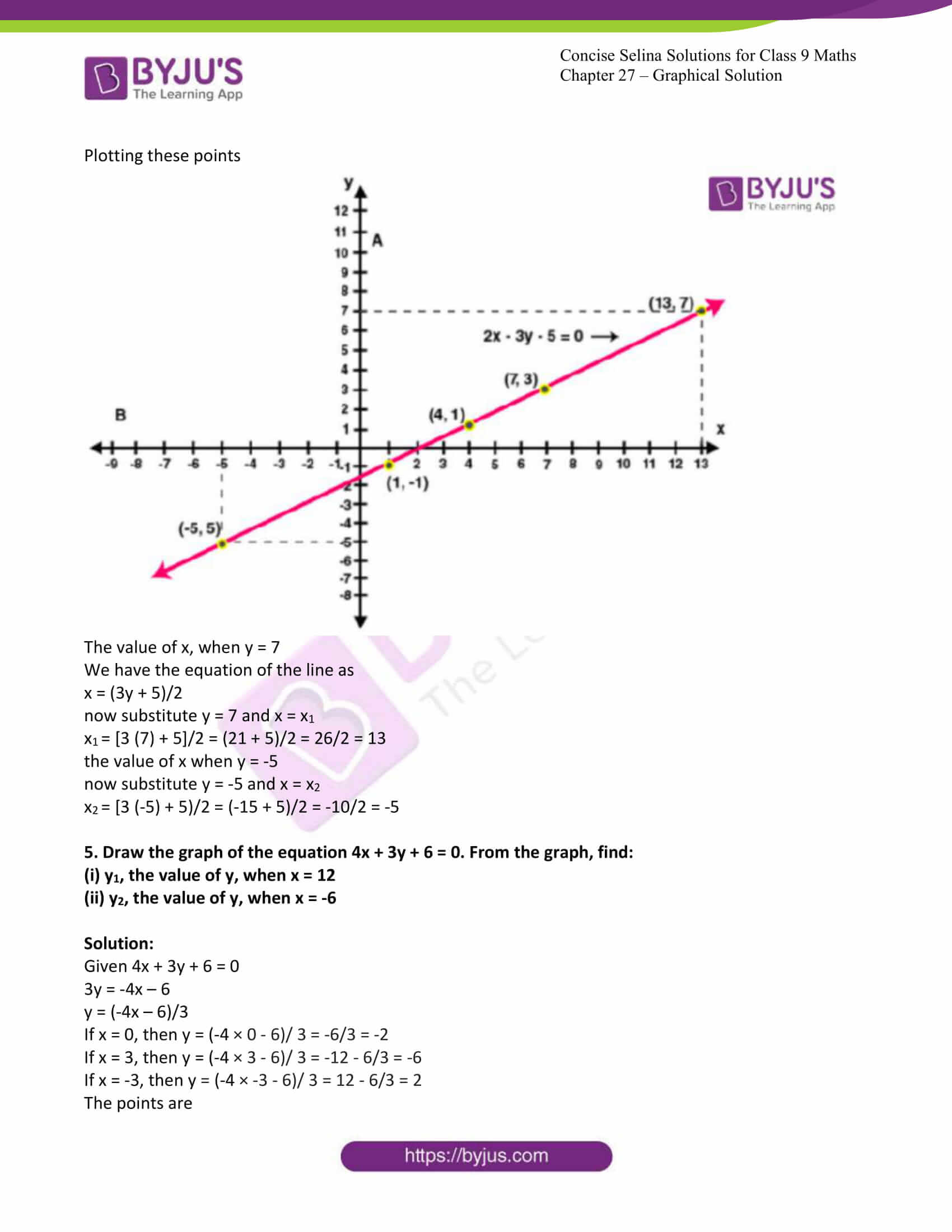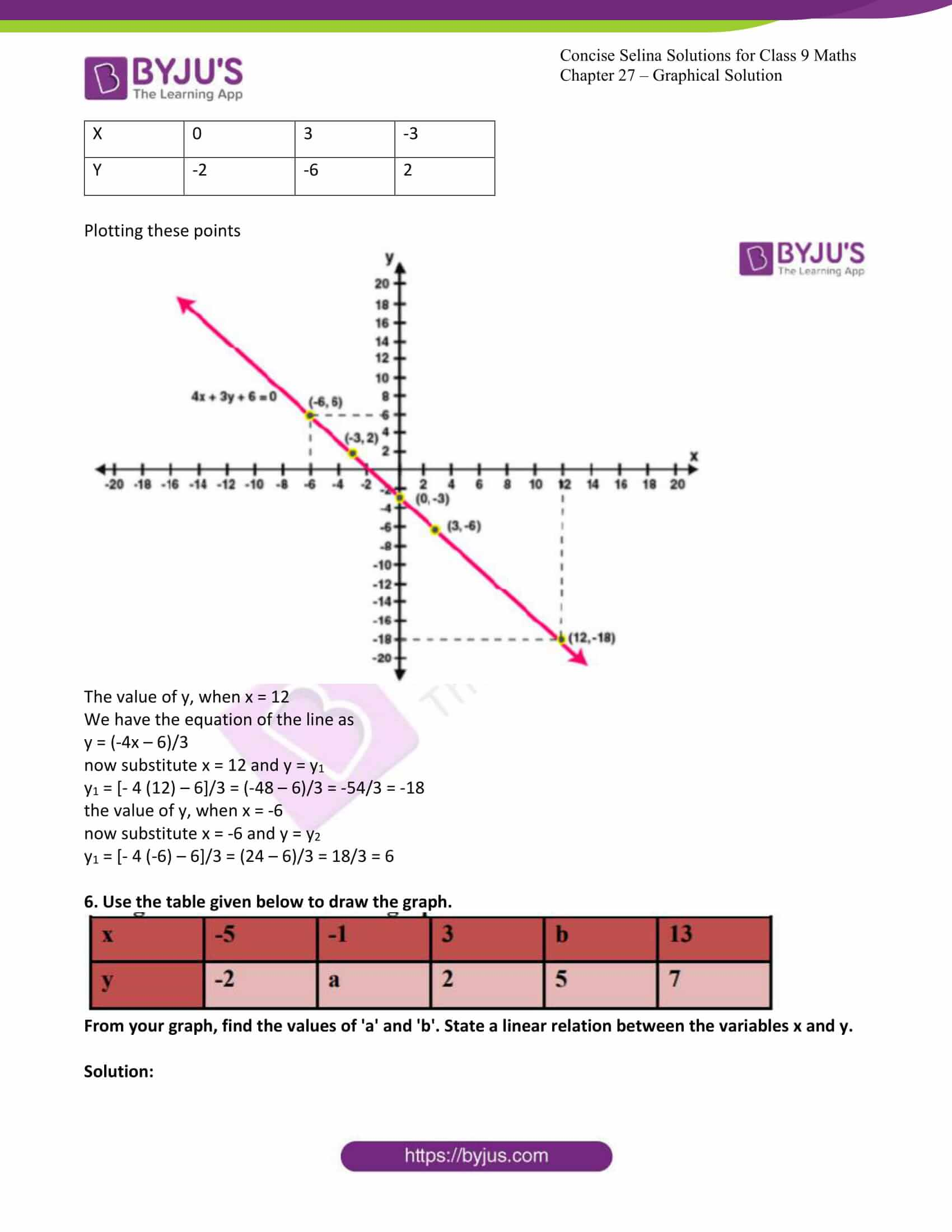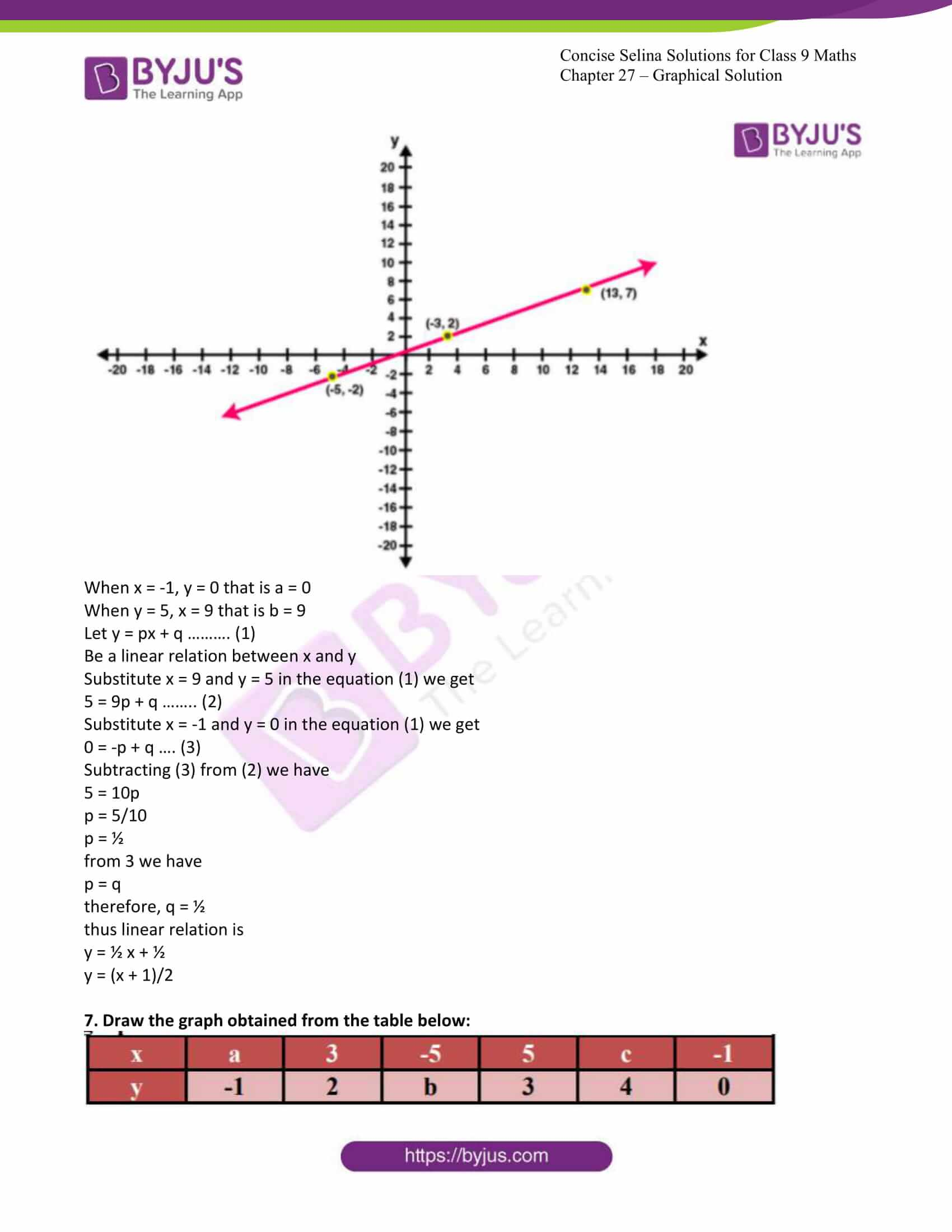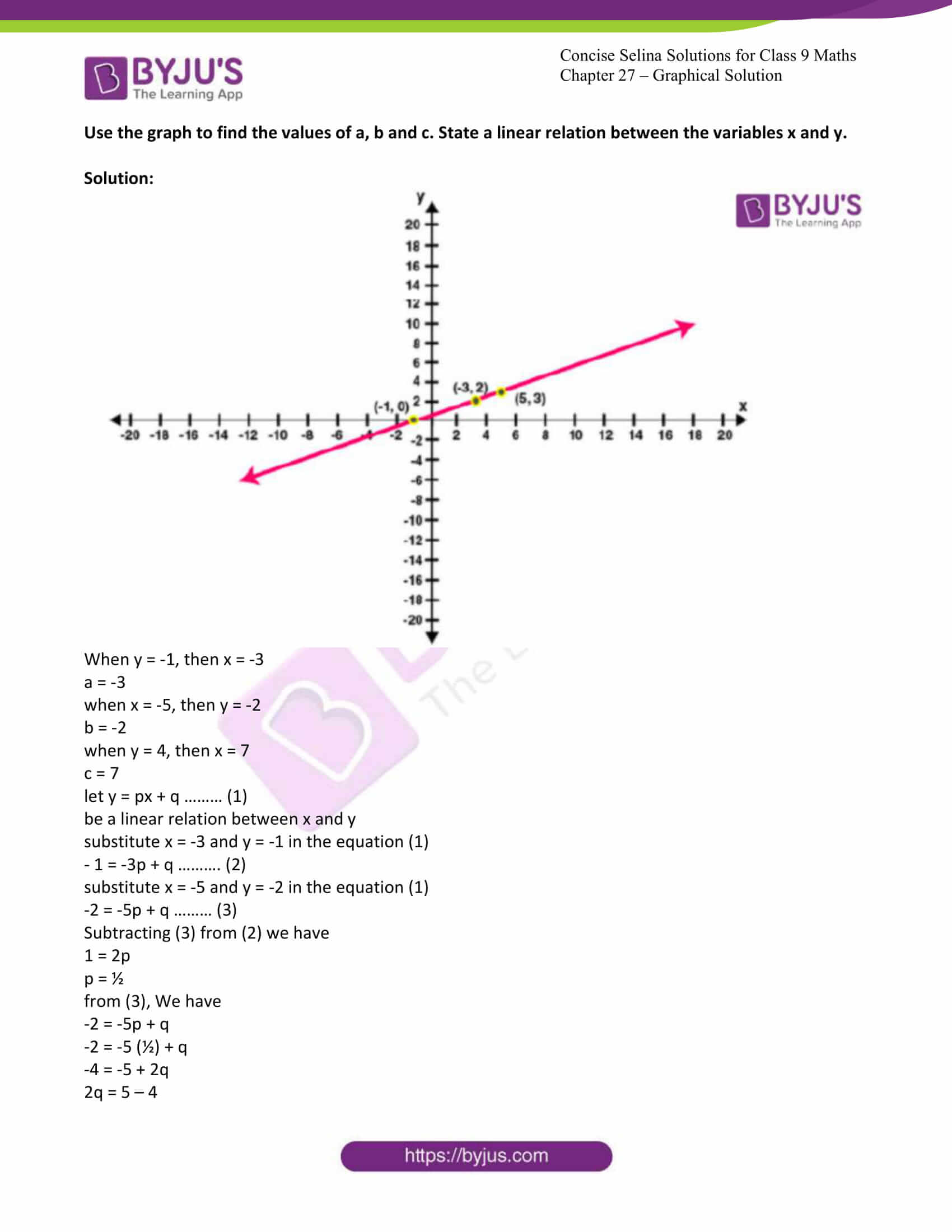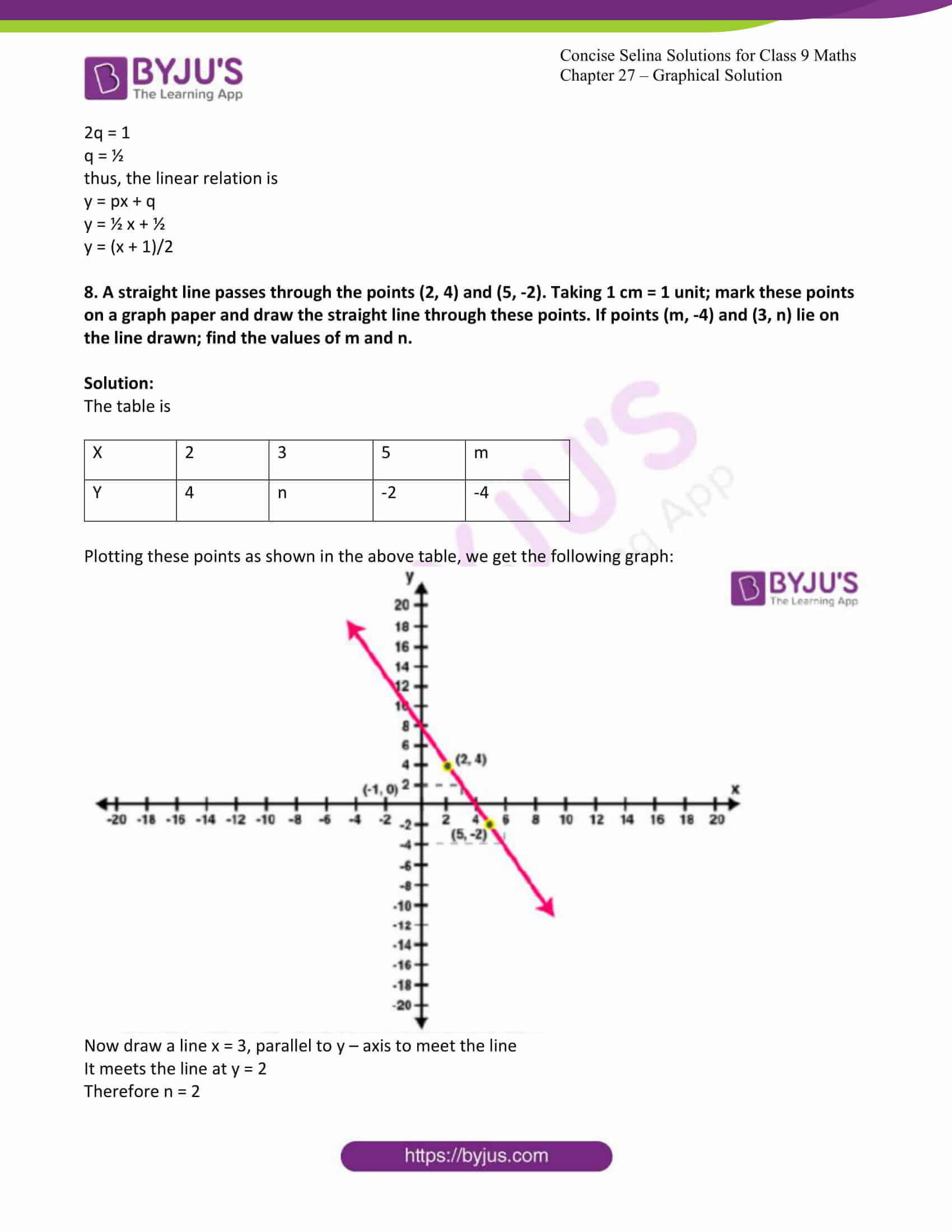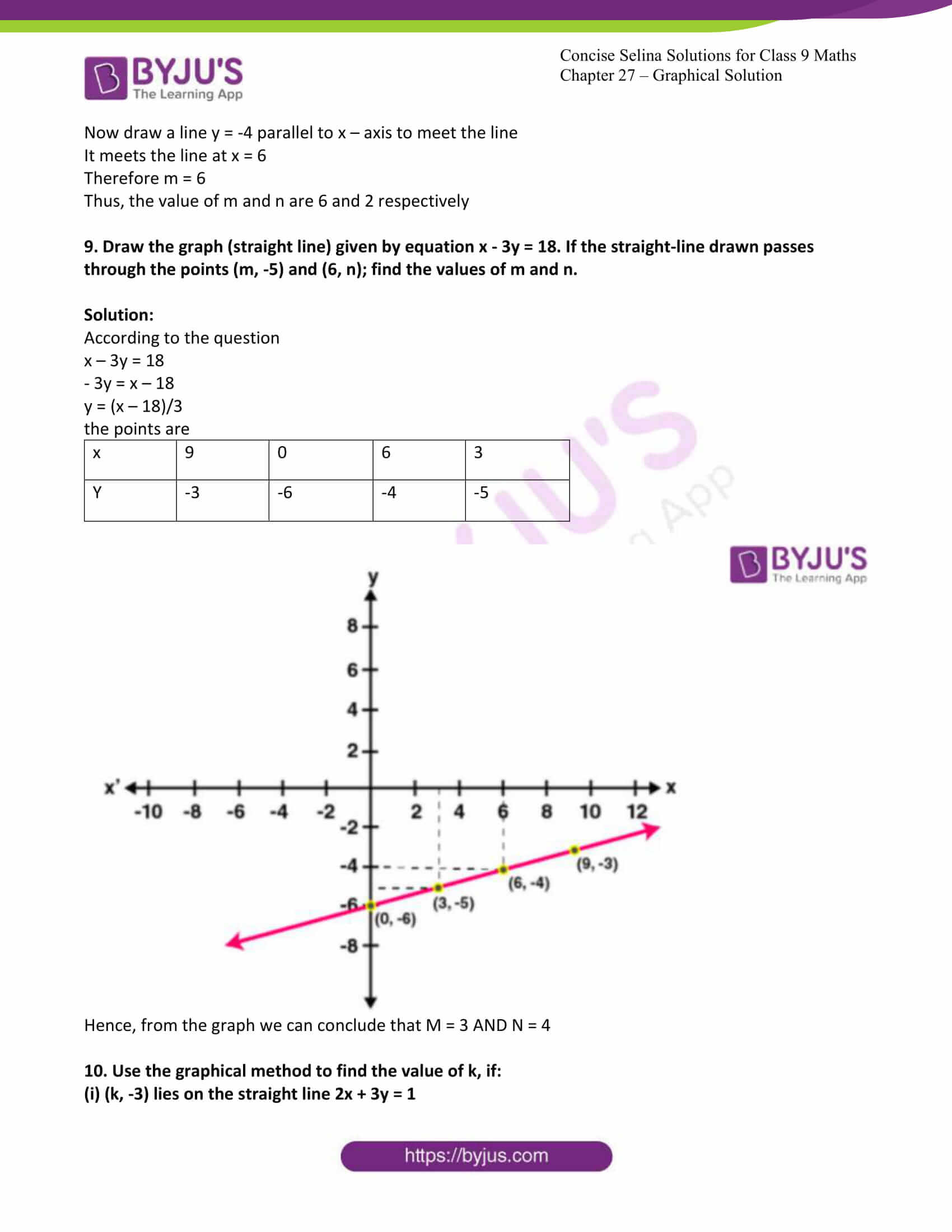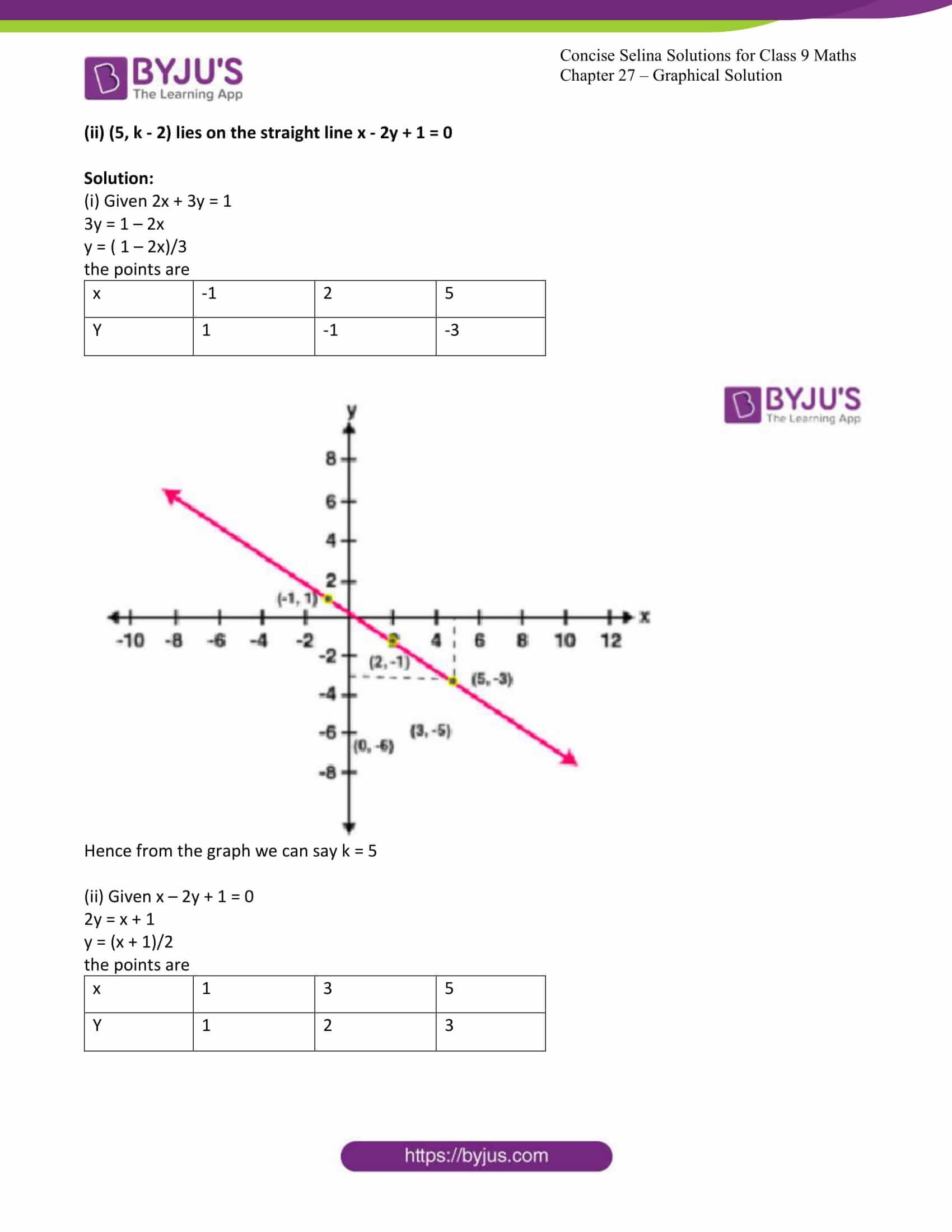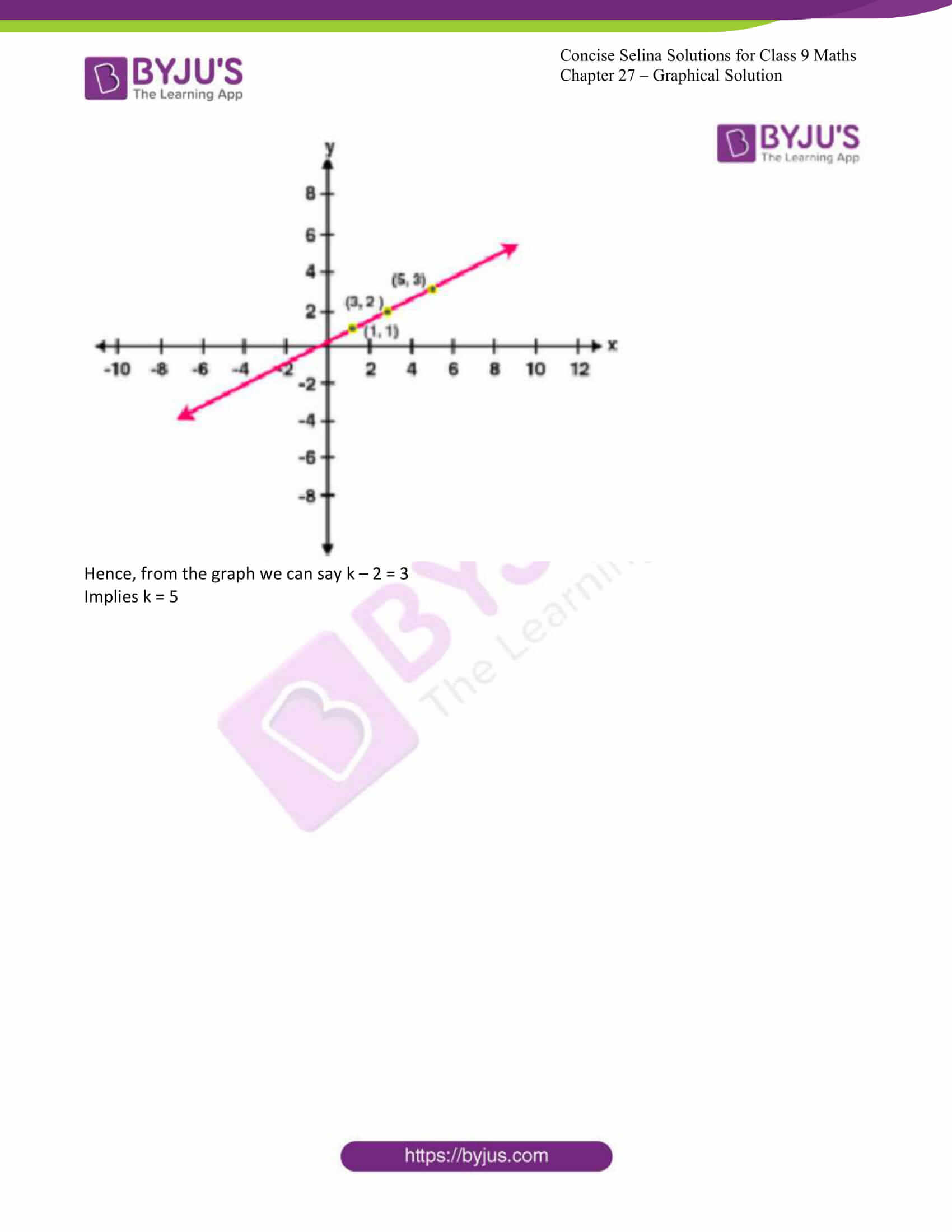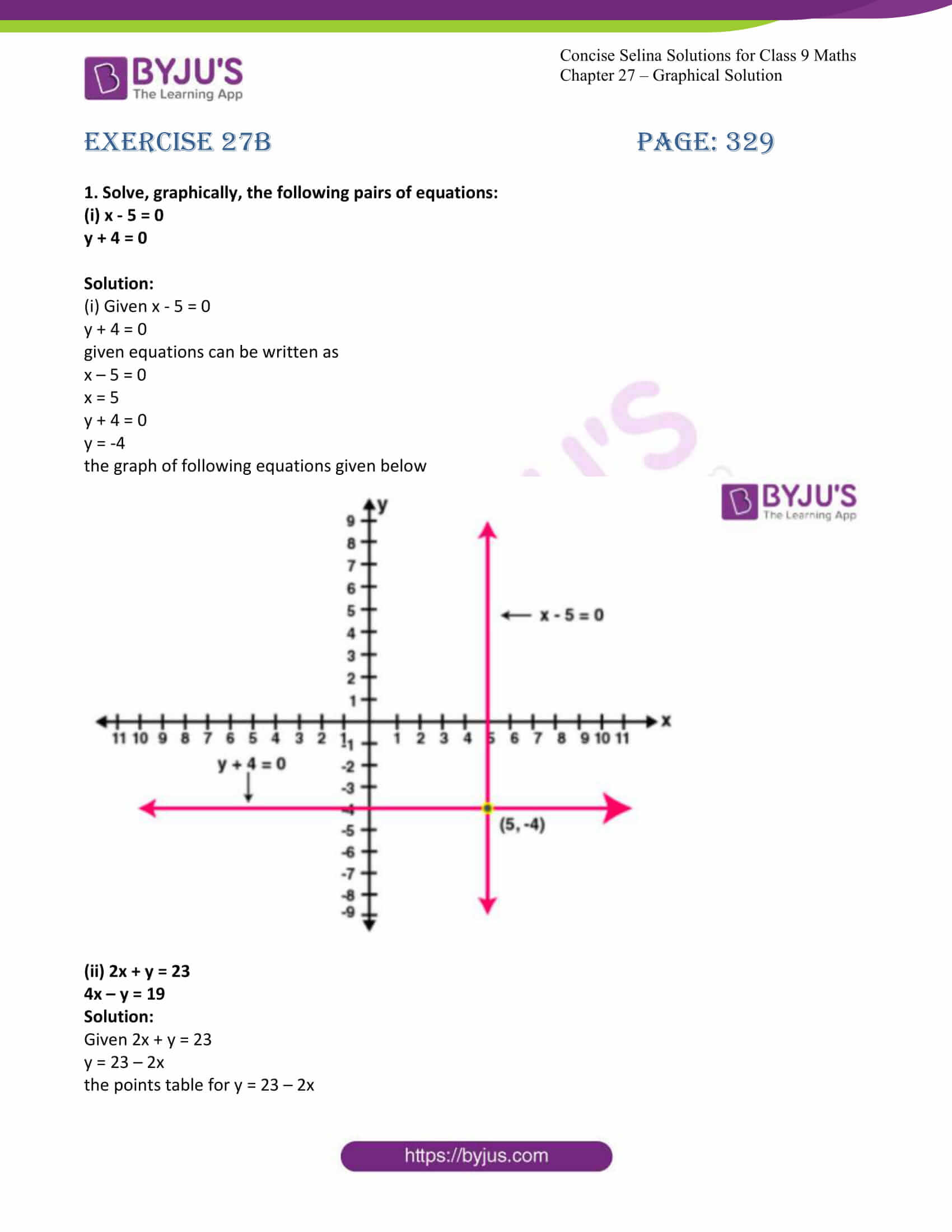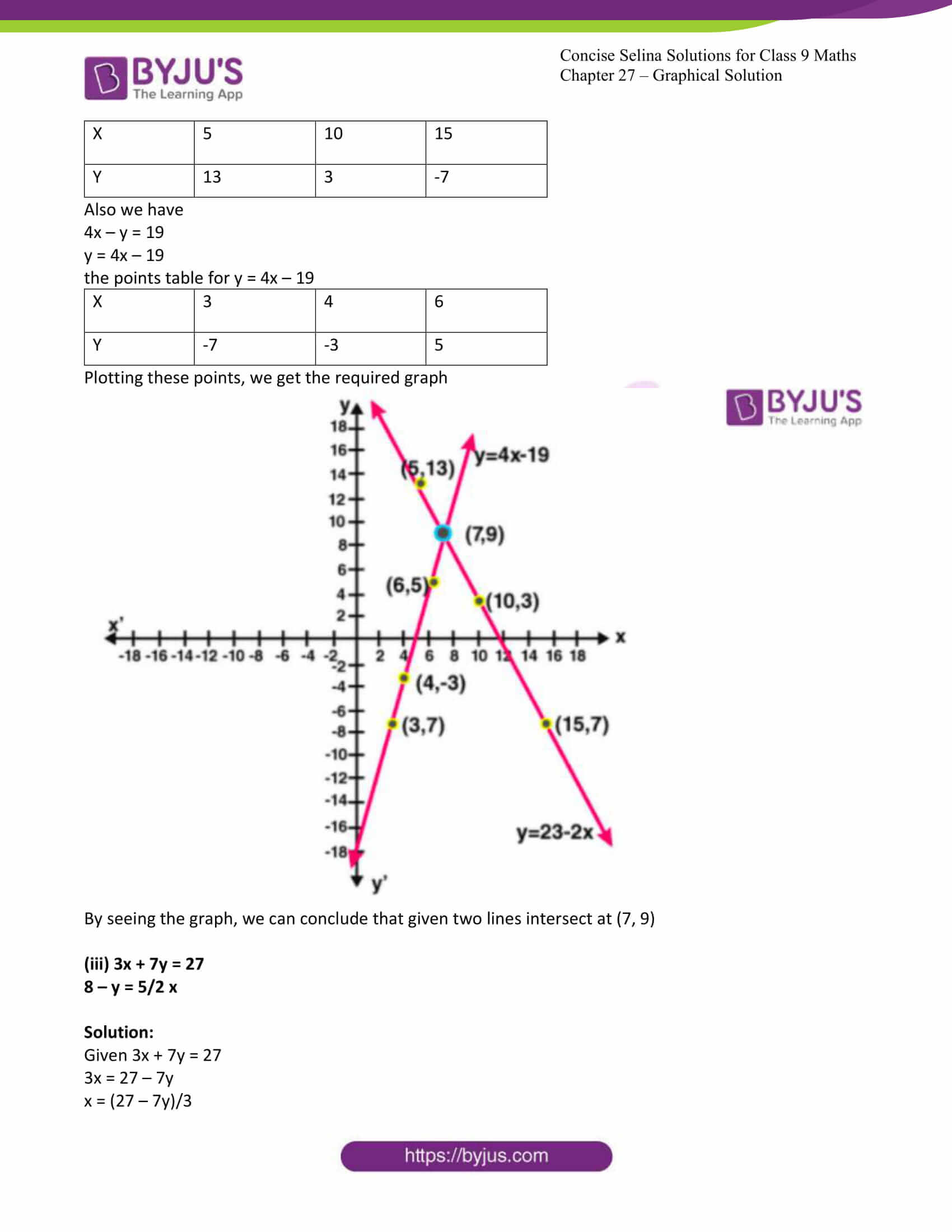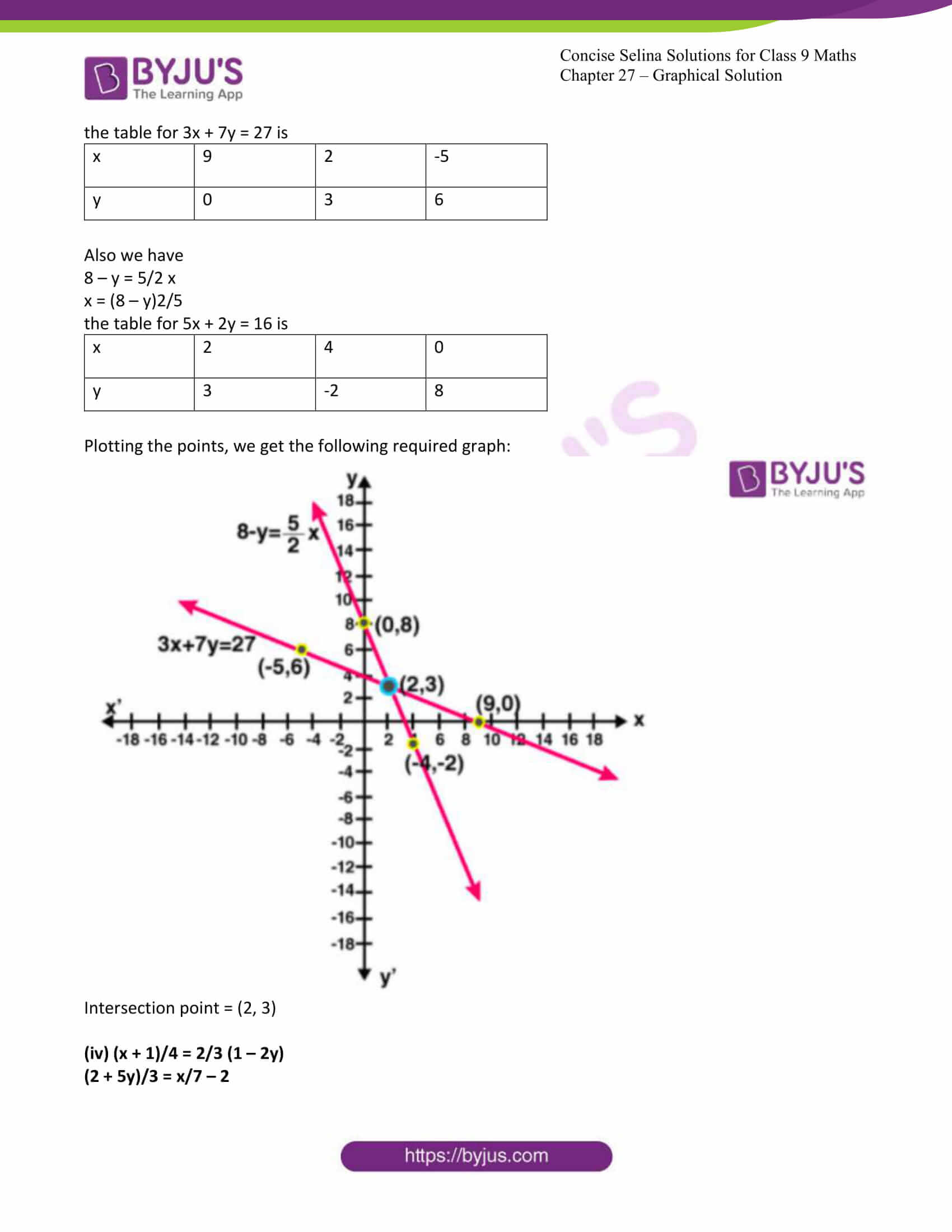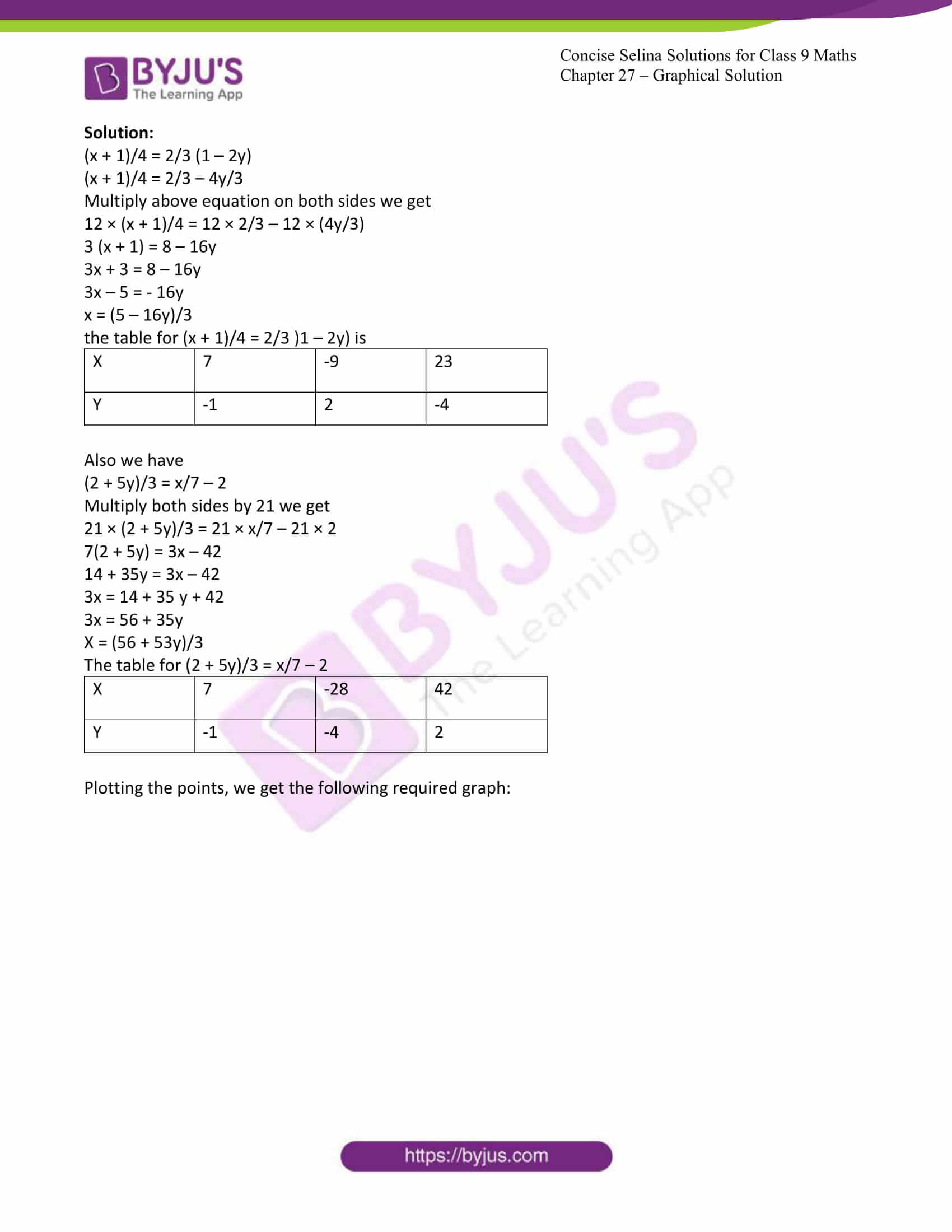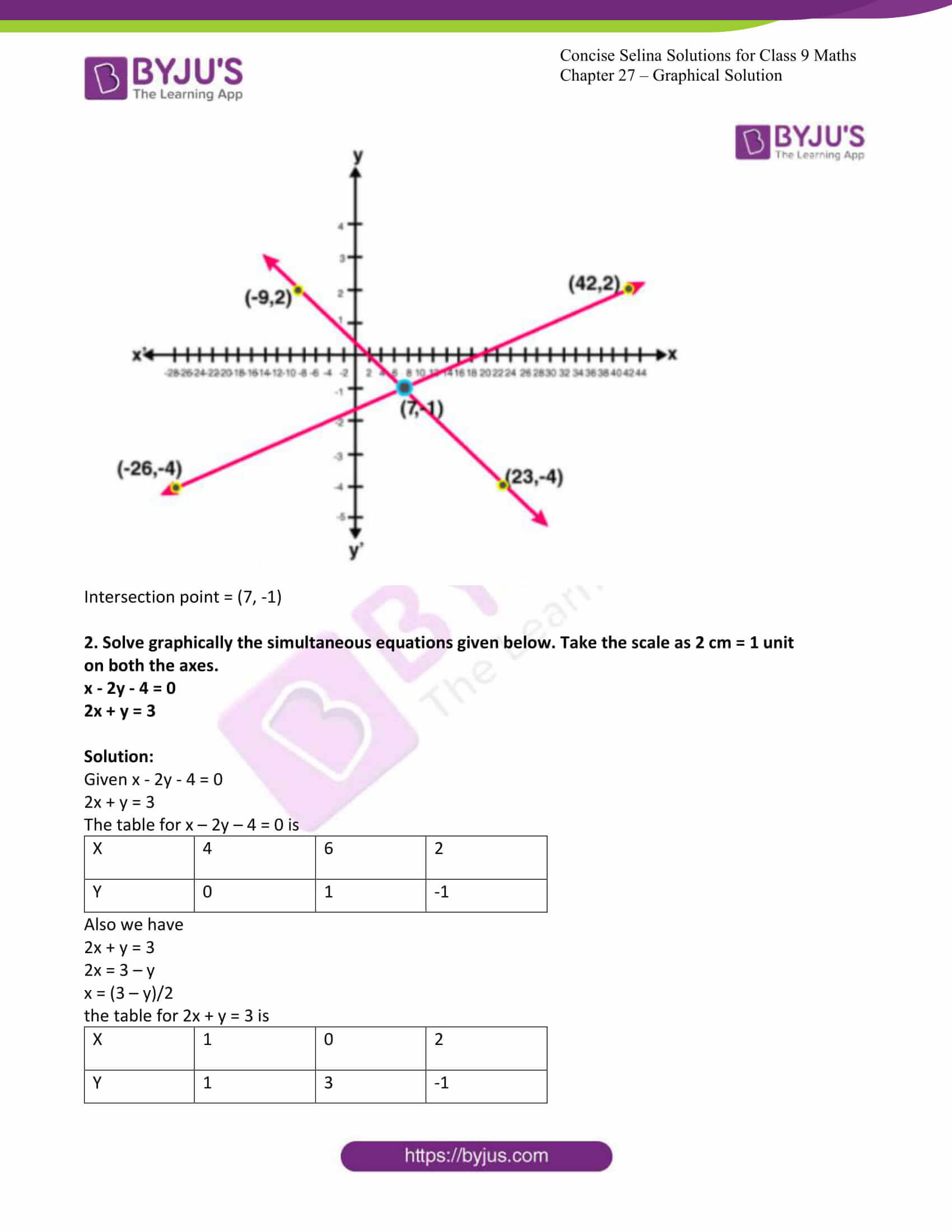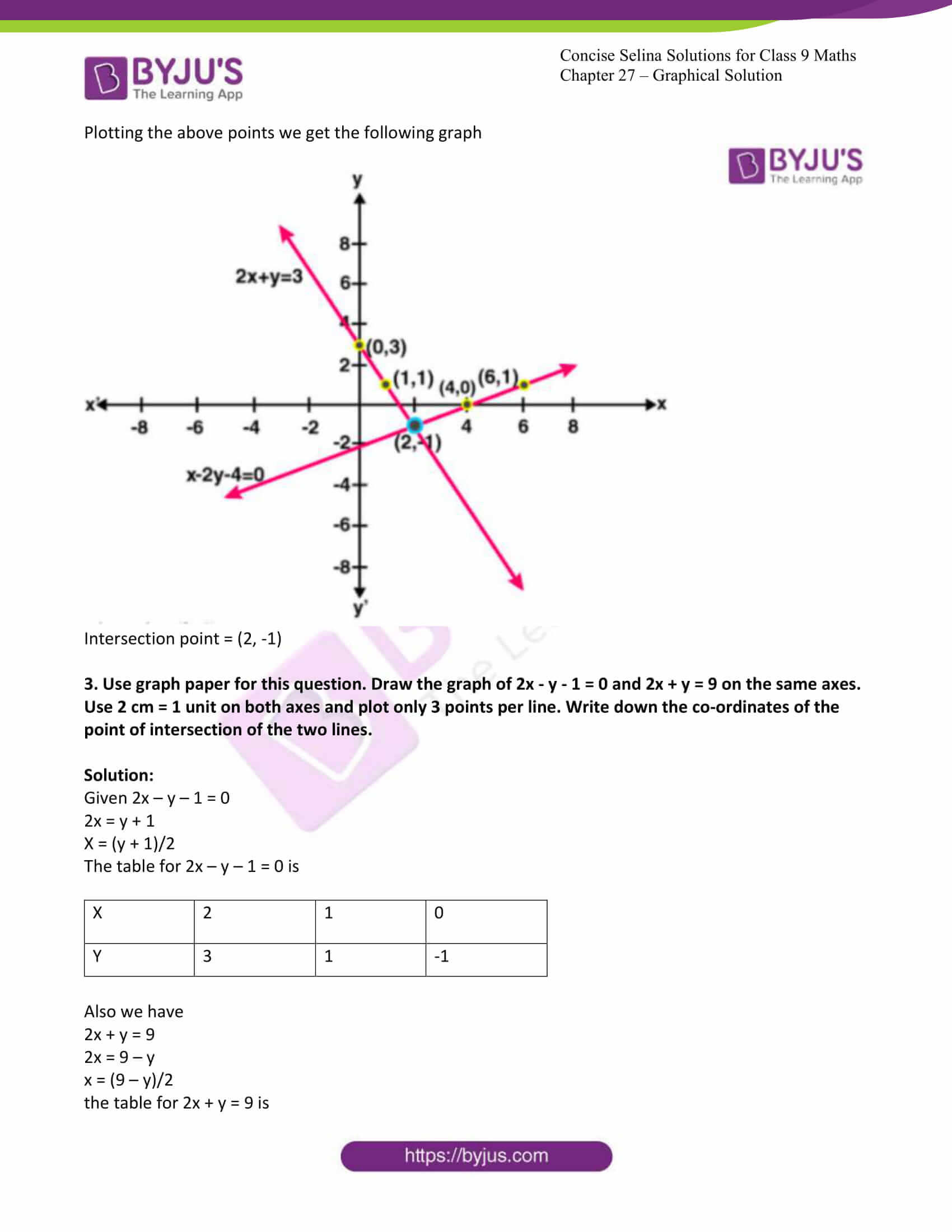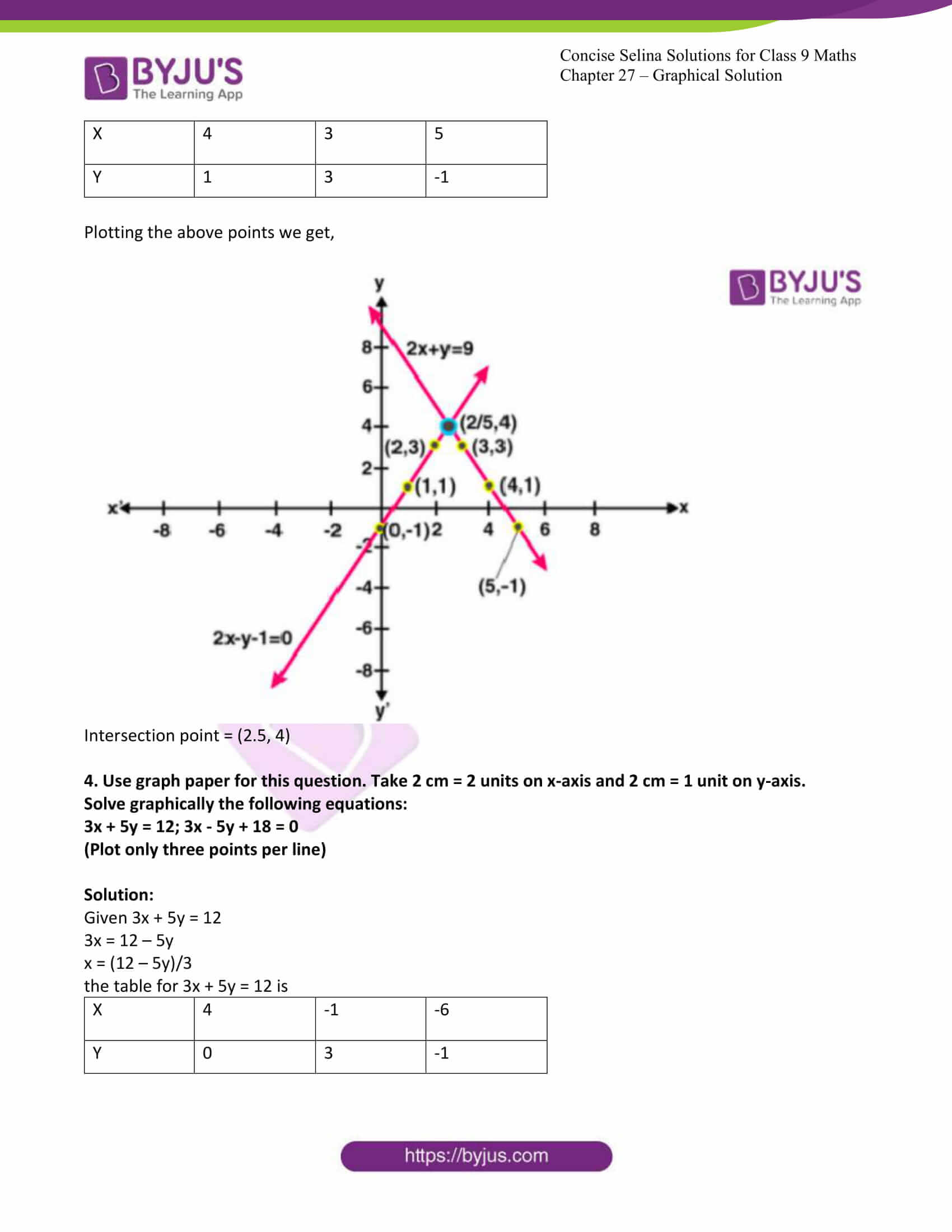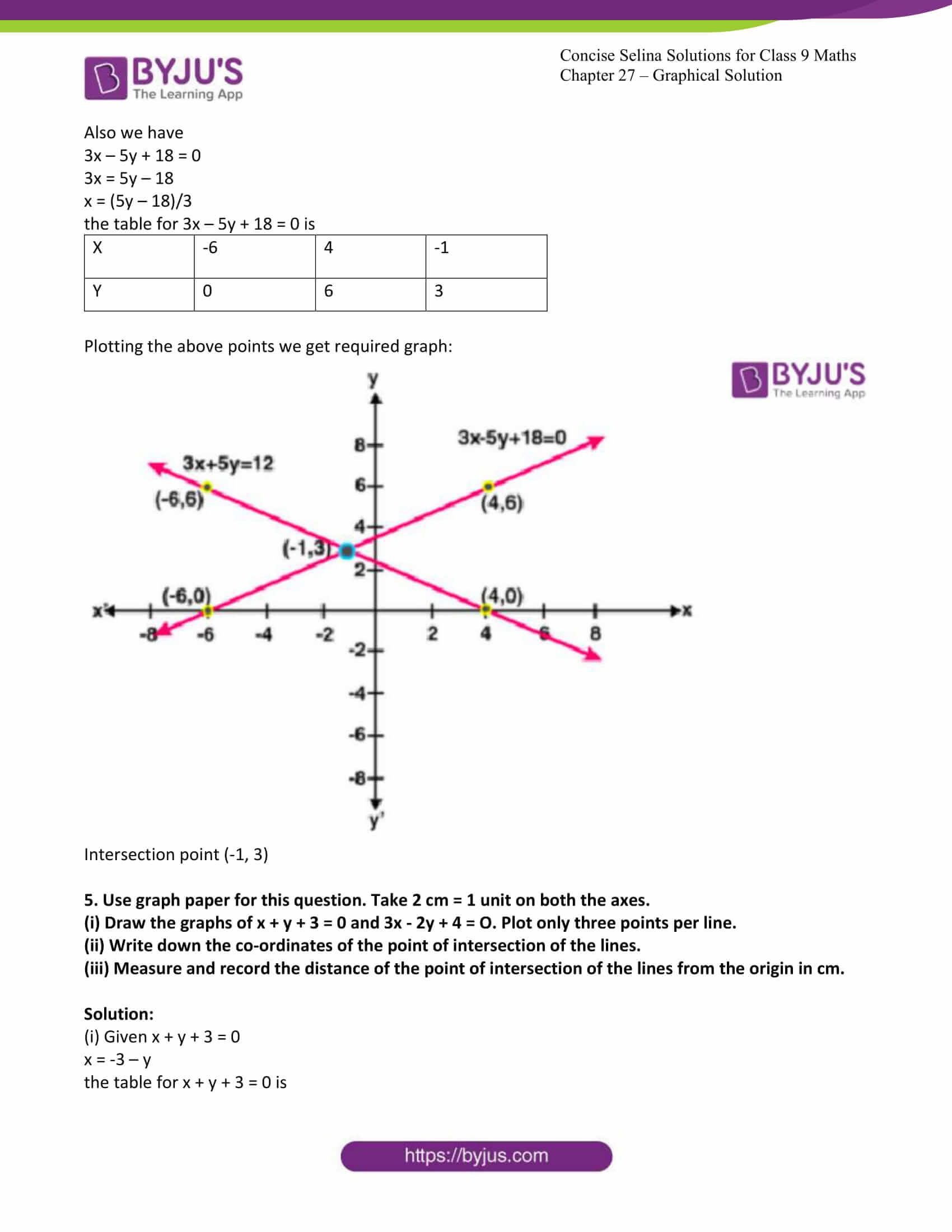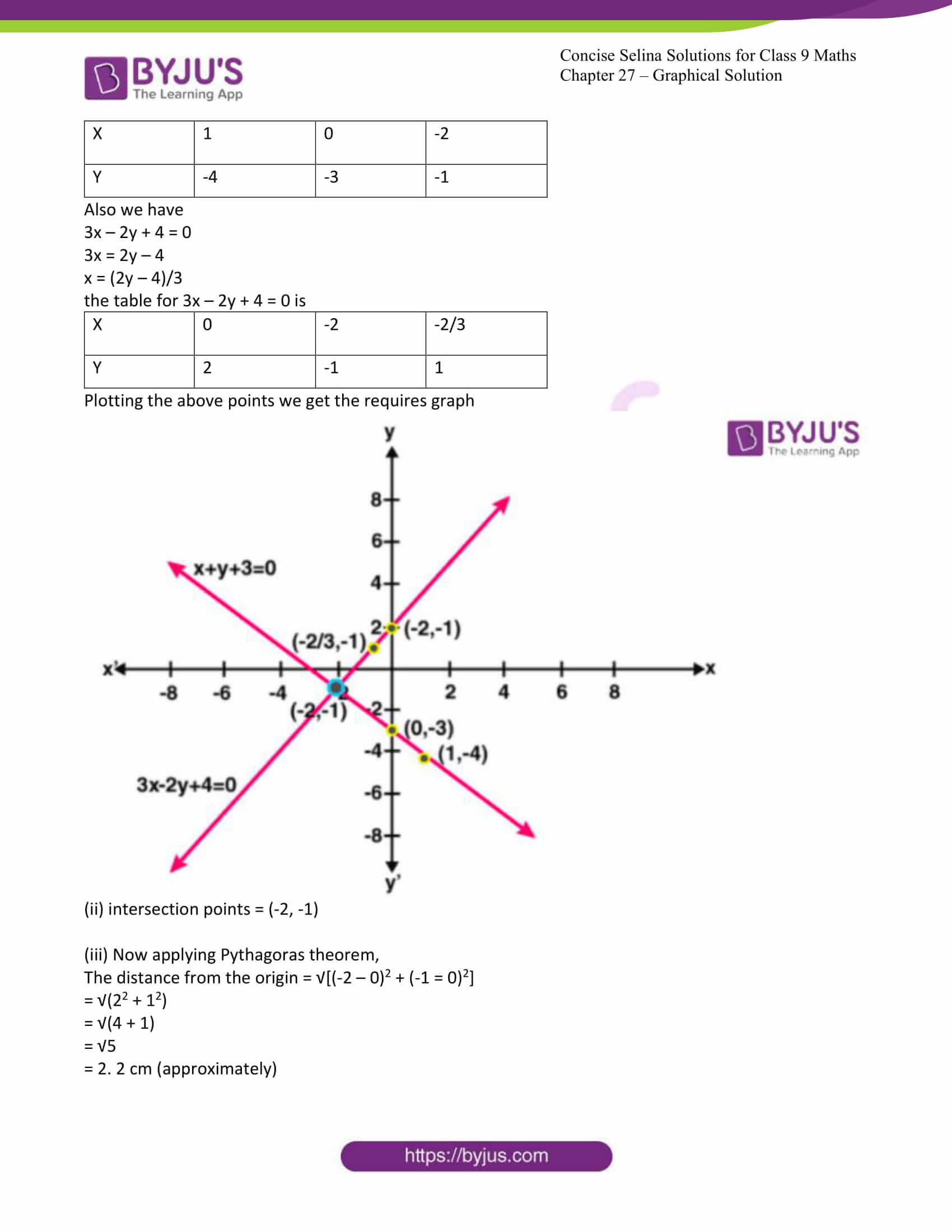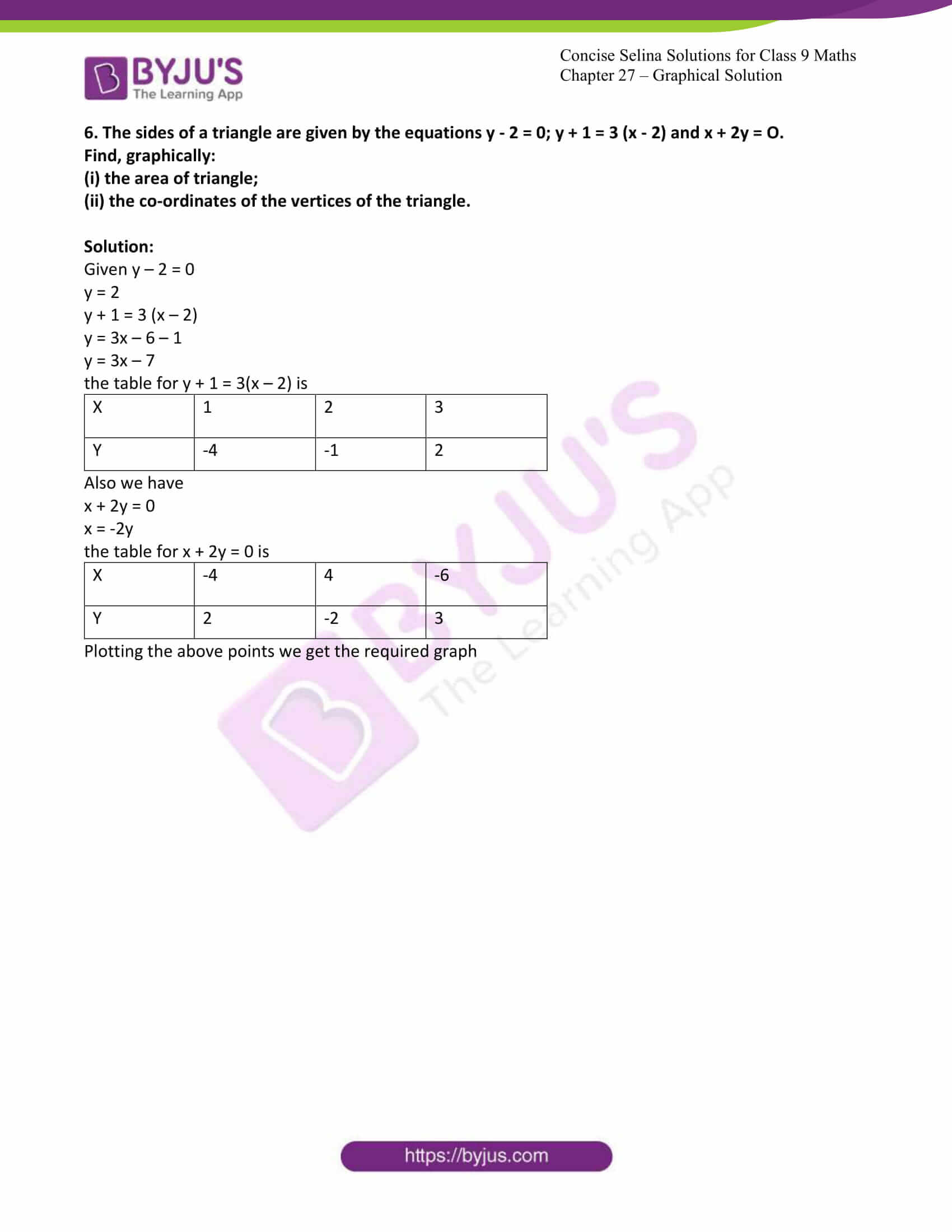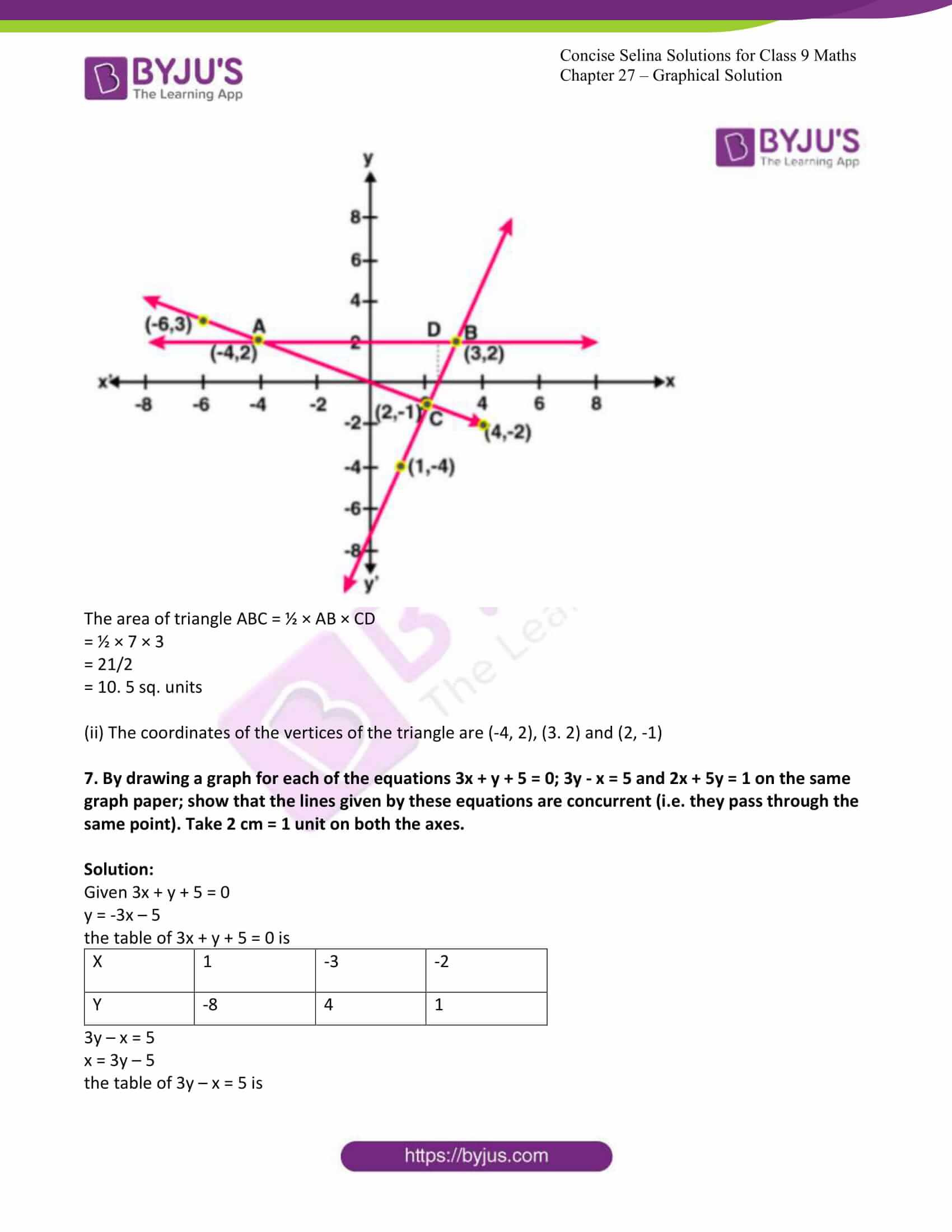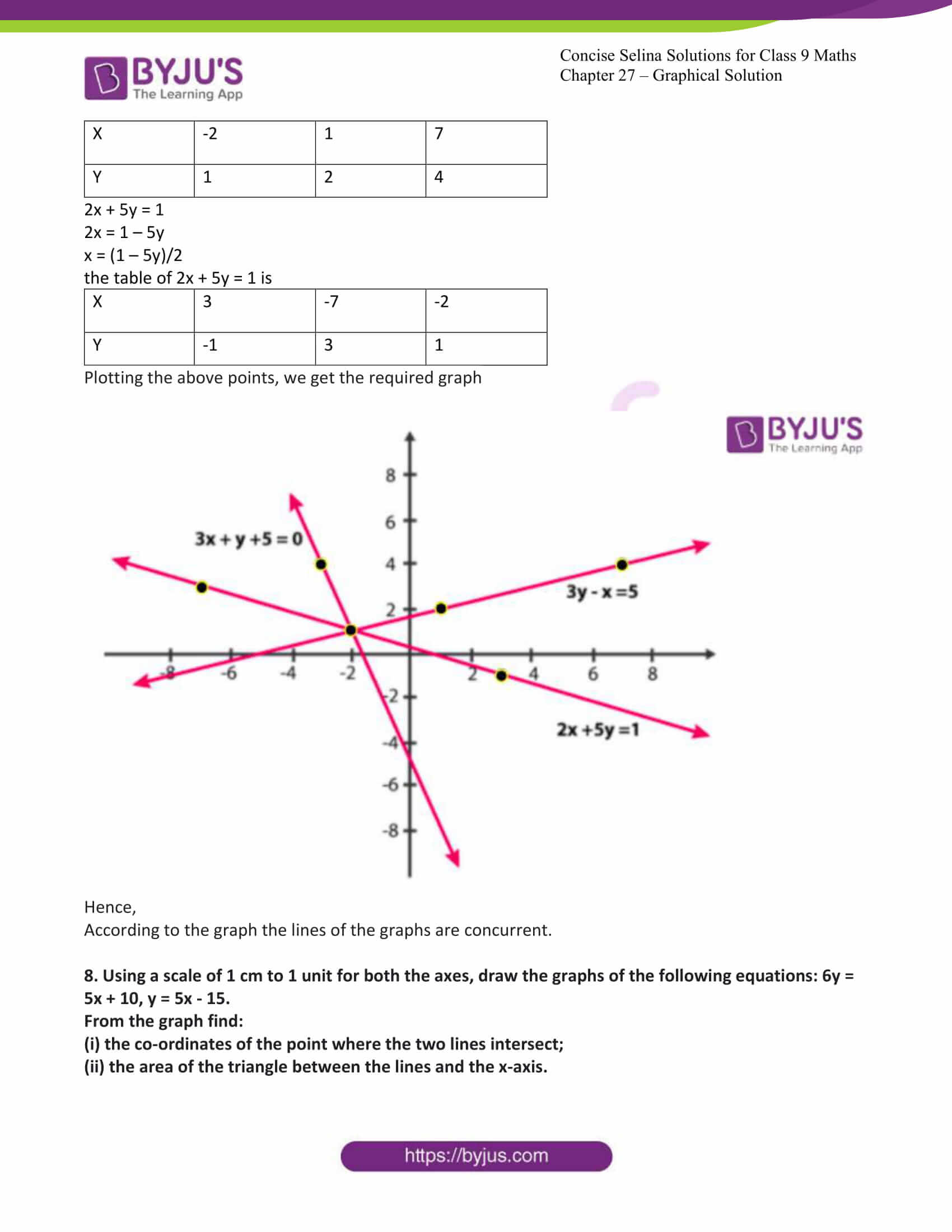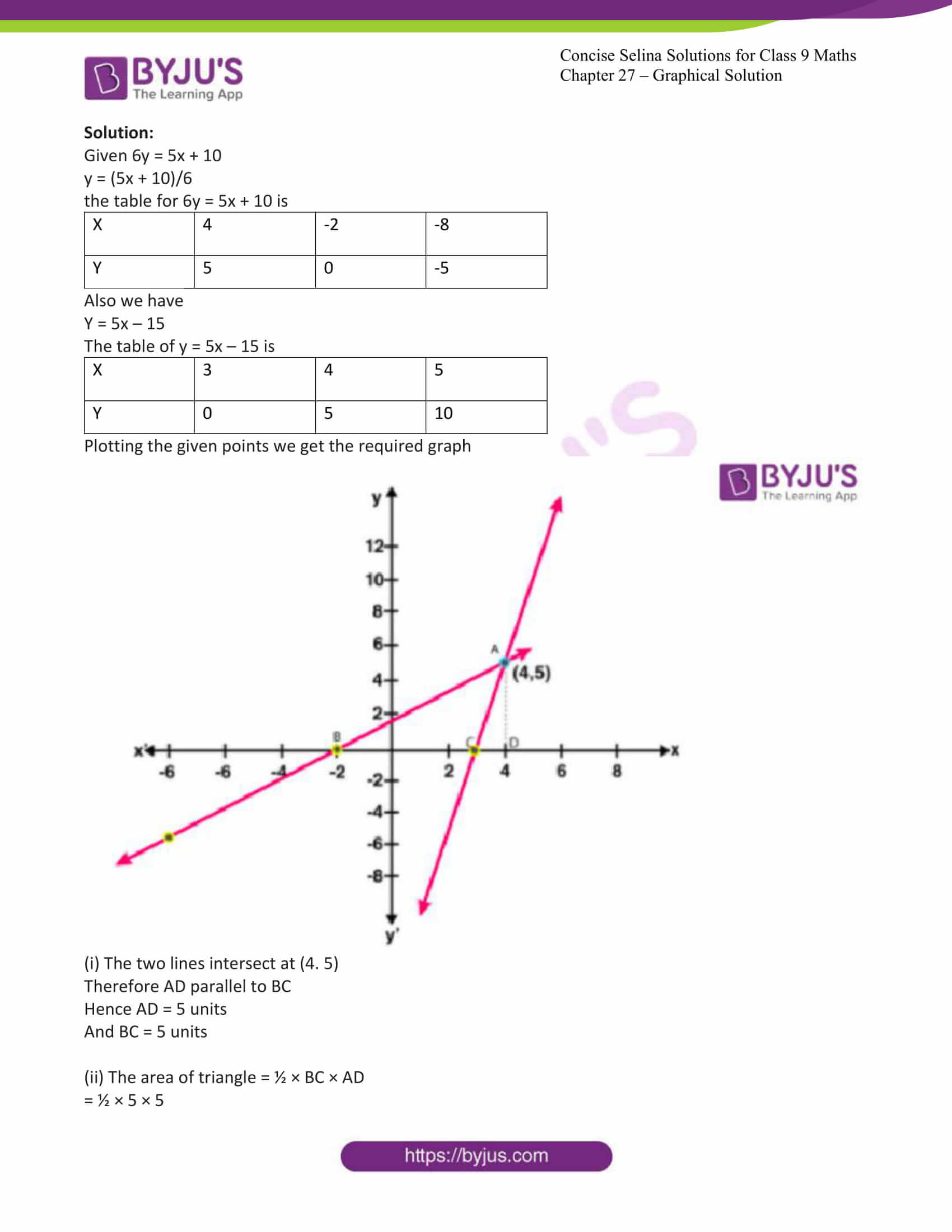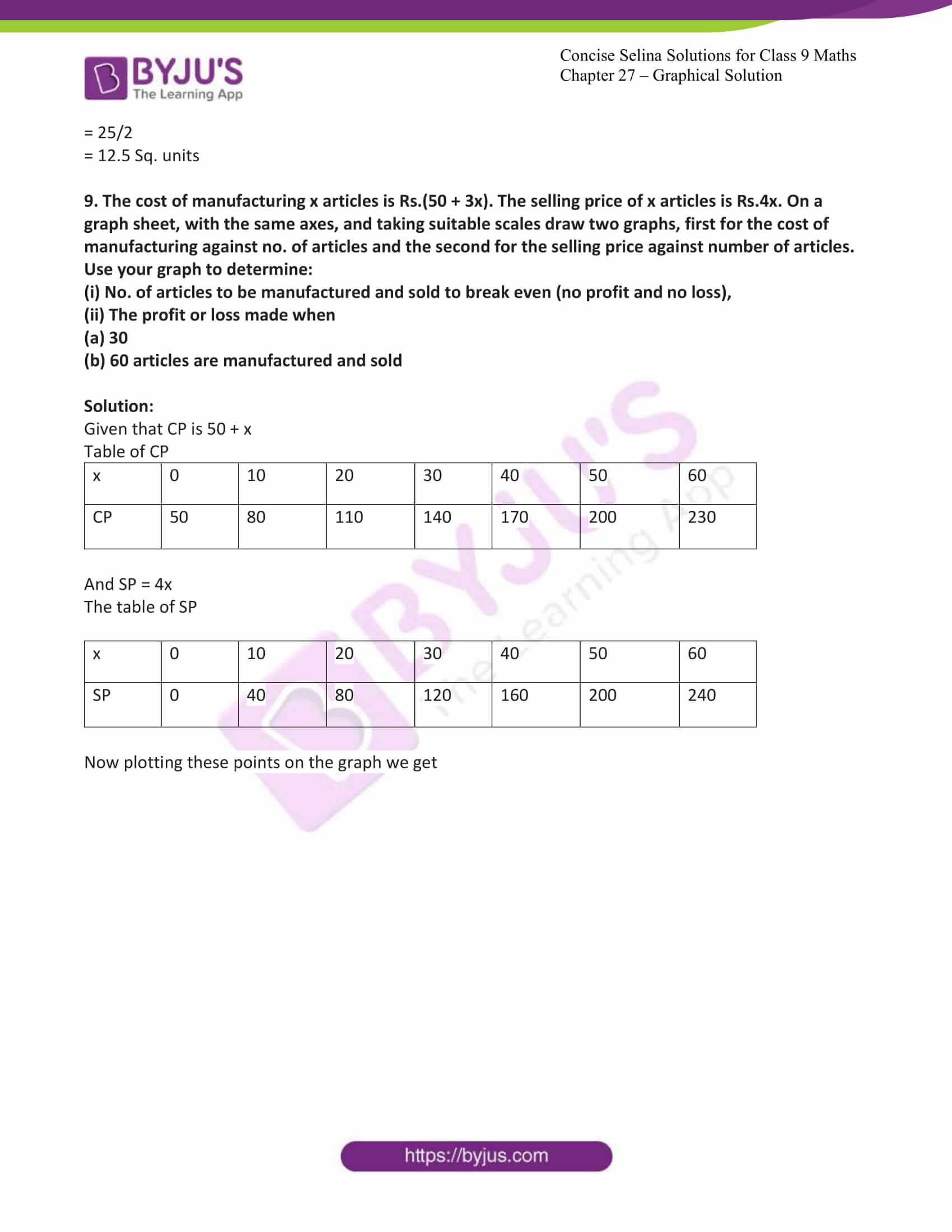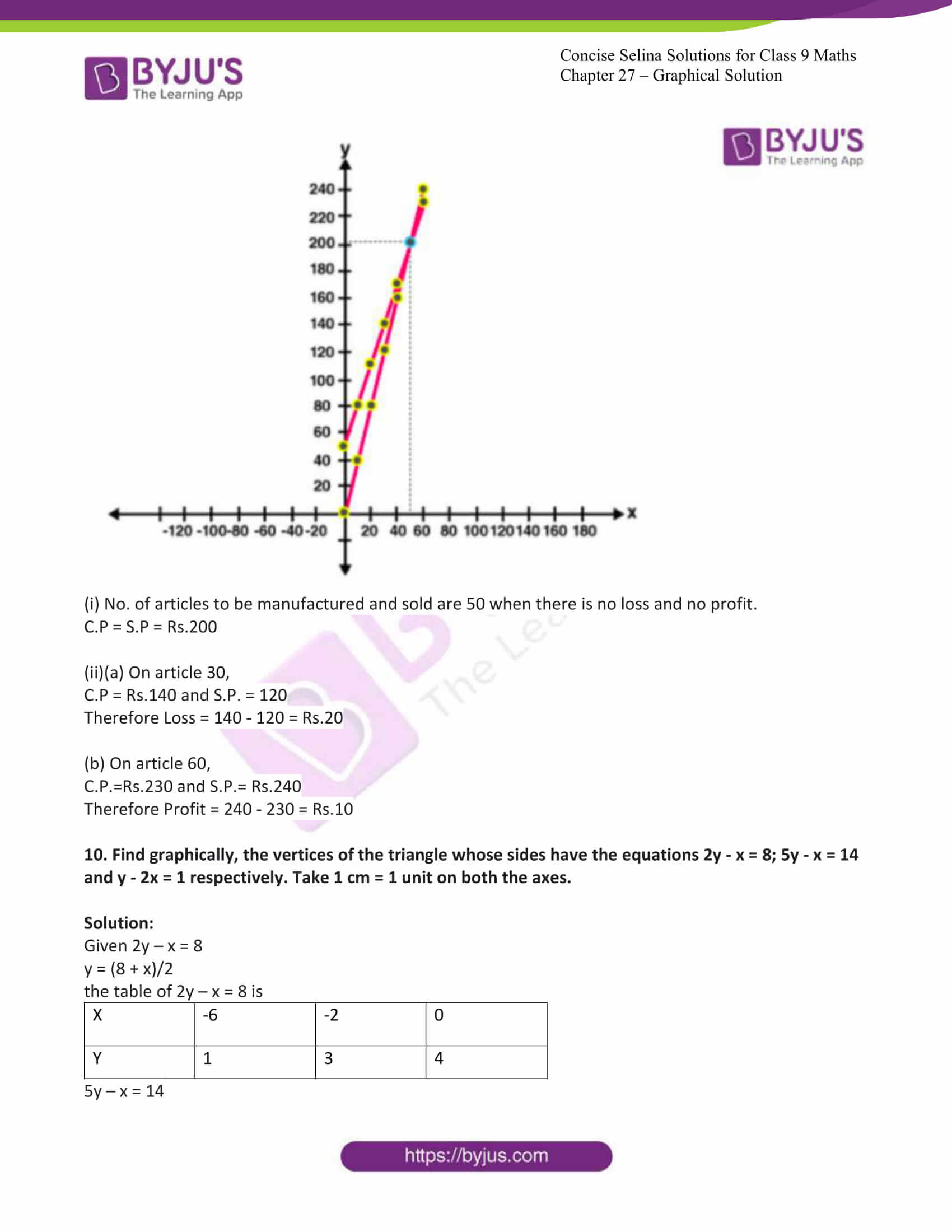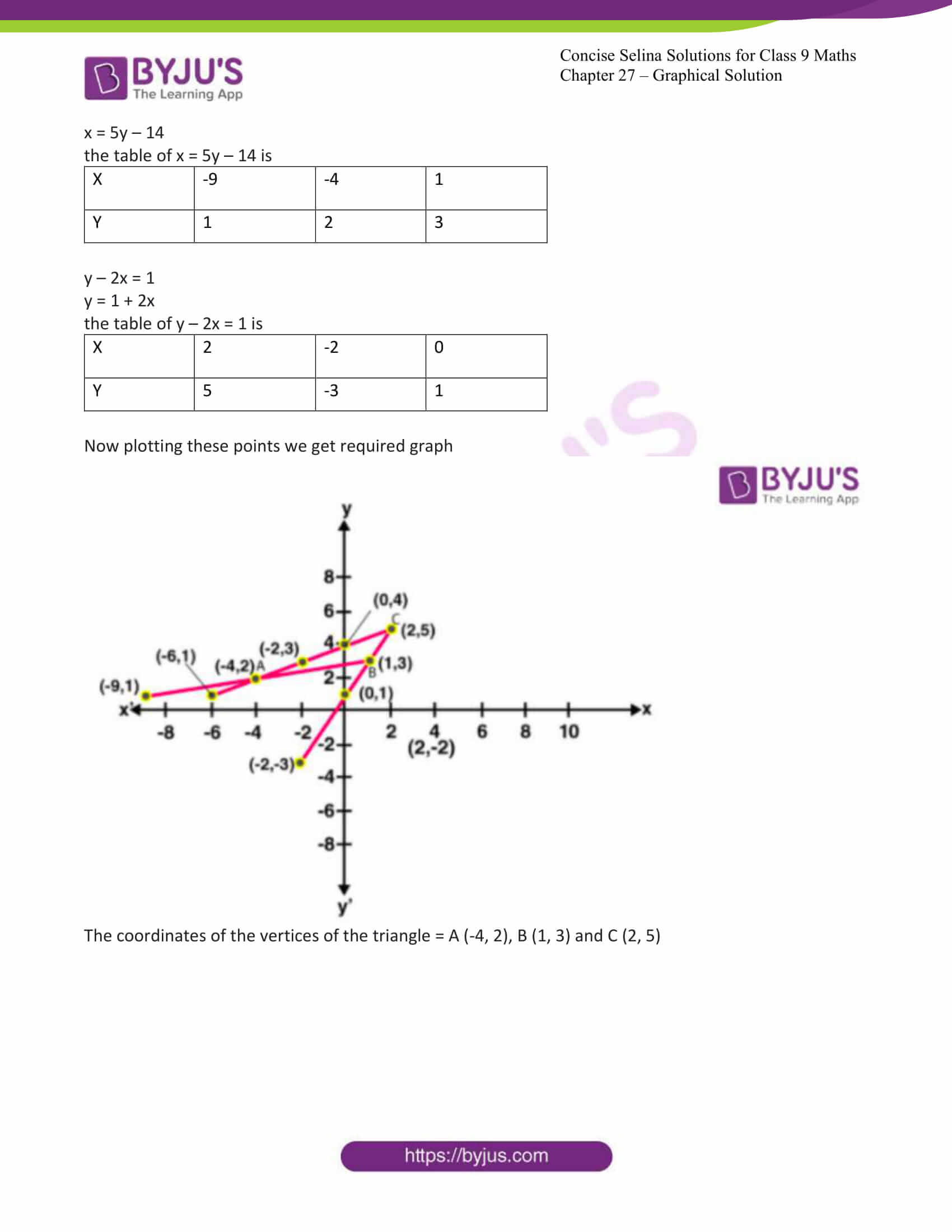Exercise 27A Page: 326

1. Draw the graph for each equation, given below:

(i) x = 5

(ii) x + 5 = 0

(iii) y = 7

(iv) y + 7 = 0

(v) 2x + 3y = 0

(vi) 3x + 2y = 6

(vii) x – 5y + 4 = 0

(viii) 5x + y + 5 = 0

Solution:

(i) x = 5

The graph x = 5 in the following figure is a straight line AB which is parallel to y axis at a distance of 5 units from it.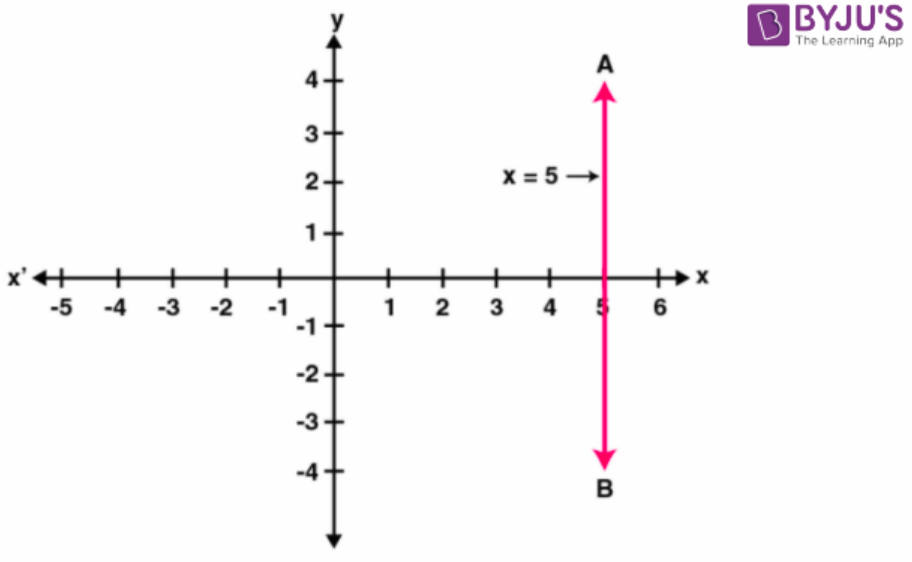(ii) x + 5 = 0

Therefore x = -5

The graph x= -5 in the following figure is a straight-line AB which is parallel to y axis at a distance of 5 units from it in the negative x direction.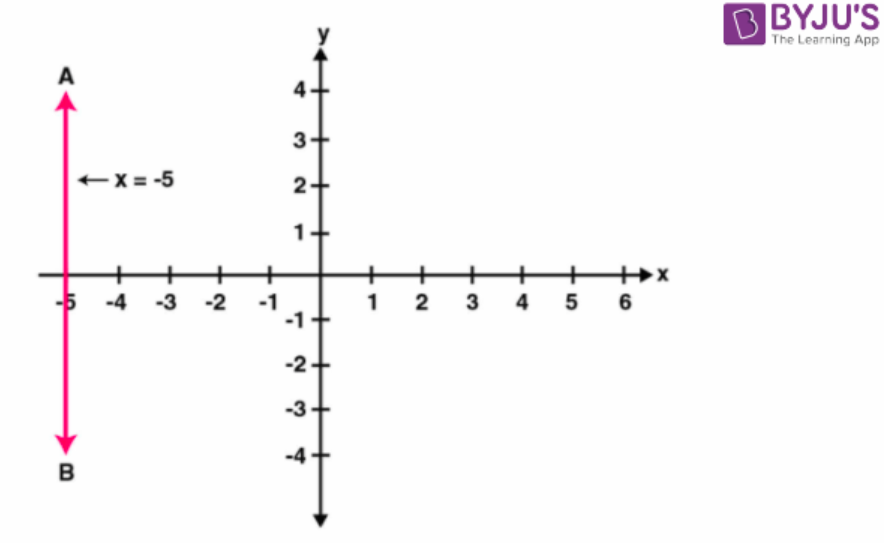(iii) y = 7

The graph y = 7 in the following figure is a straight line AB which is parallel to x axis at a distance of 7 units from it.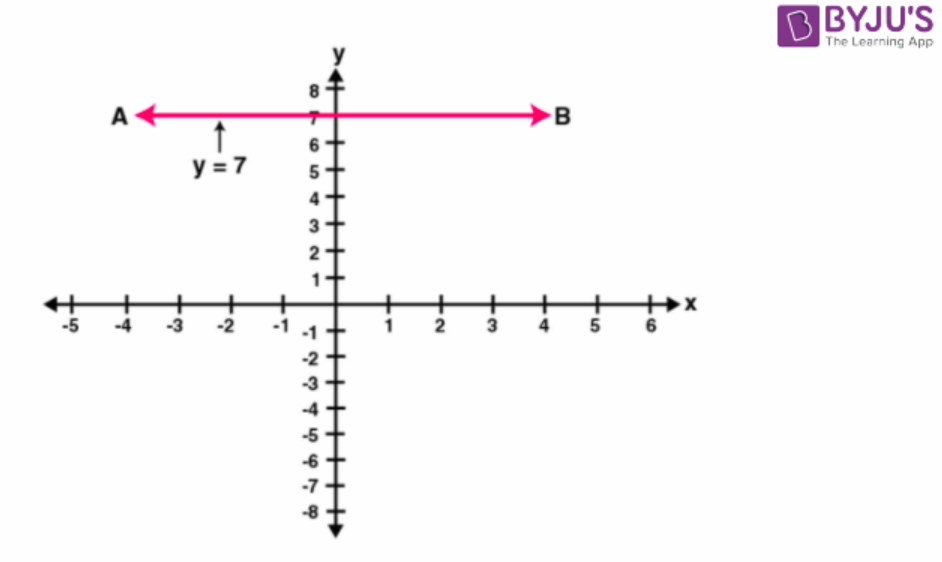(iv) y + 7 = 0

y = -7

The graph y = -7 in the following figure is a straight line AB which is parallel to x axis at a distance of 7 units from it in the negative y direction.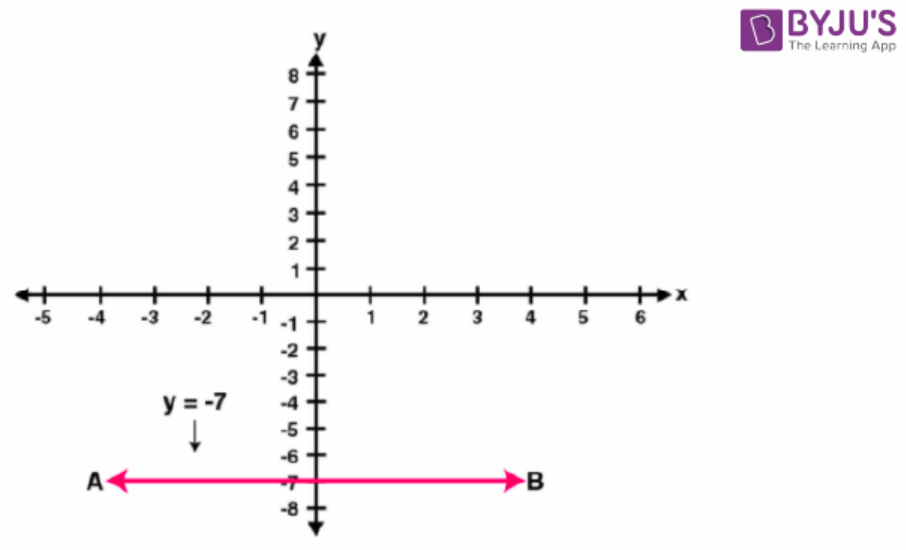(v) Given 2x + 3y = 0

3y = -2x

Therefore, y = -2x/3

If x = -3, then y = -2(-3)/3 = 6/3 = 2

If x = 3, then y = -2(3)/3 = -6/3 = -2

If x = 6, then y = -2(6)/3 = -12/3 = -4

The points are

 x -3 3 6 y 2 -2 -4

Plotting these points, we get the required graph as given below: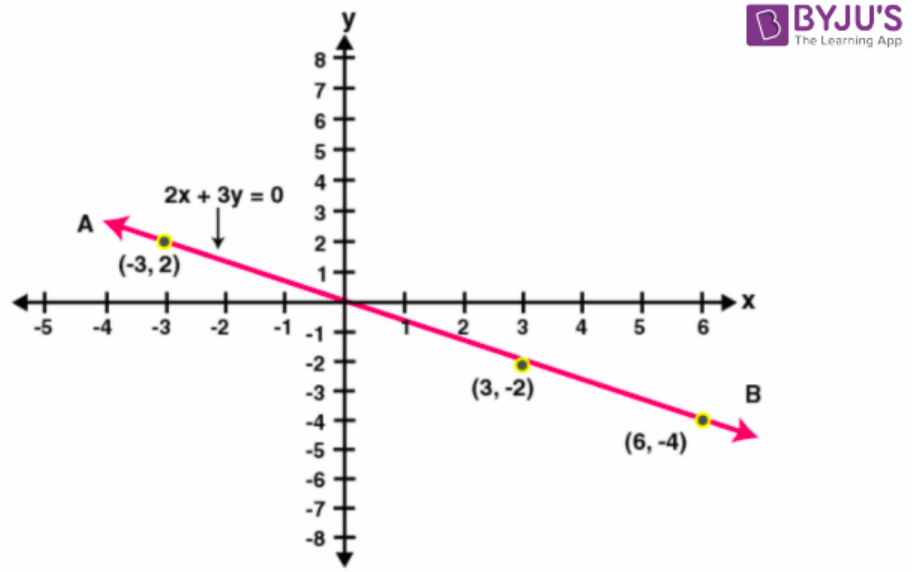(vi) Given 3x + 2y = 6

2y = 6 – 3x

Therefore, y = (6 – 3x)/2

If x = 0, then y = (6 – 3 × 0)/2 = 6 – 0/2 = 3

If x = 2, then y = (6 – 3 × 2)/2 = 6 – 6/2 = 0

If x = 4, then y = (6 – 3 × 4)/2 = 6 – 12/2 = -3

The points are

 x 0 2 4 y 3 0 -3

Plotting these points, we get the required graph as given below: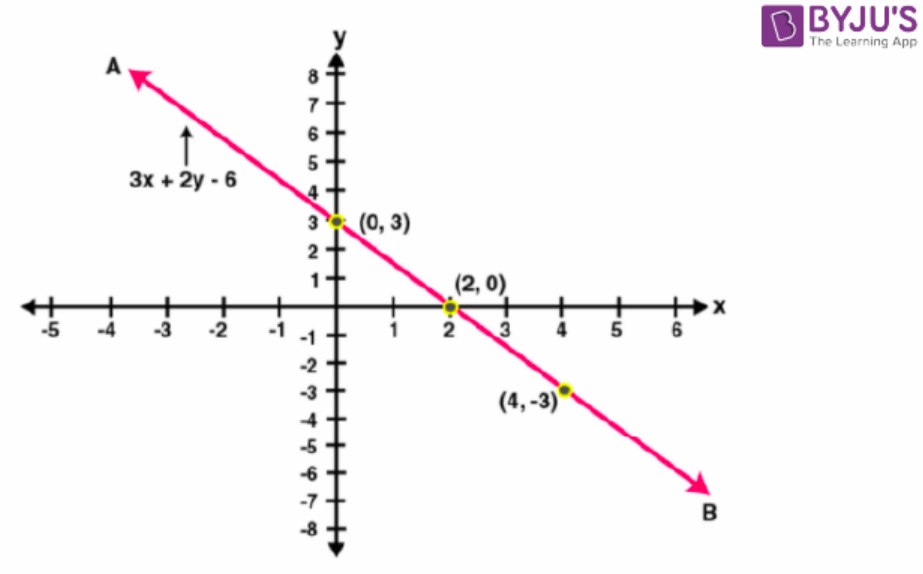(vii) Given x – 5y + 4 = 0

5y = 4 + x

Therefore, y = 4 + x/5

If x = 1, then y = (1 + 4)/5 = 5/5 = 1

If x = 6, then y = (6 + 4)/5 = 10/5 = 2

If x = -4, then y = (-4 + 4)/5 = 0/5 = 0

The points are

 x 1 6 -4 y 1 2 0

Plotting these points, we get the required graph as given below: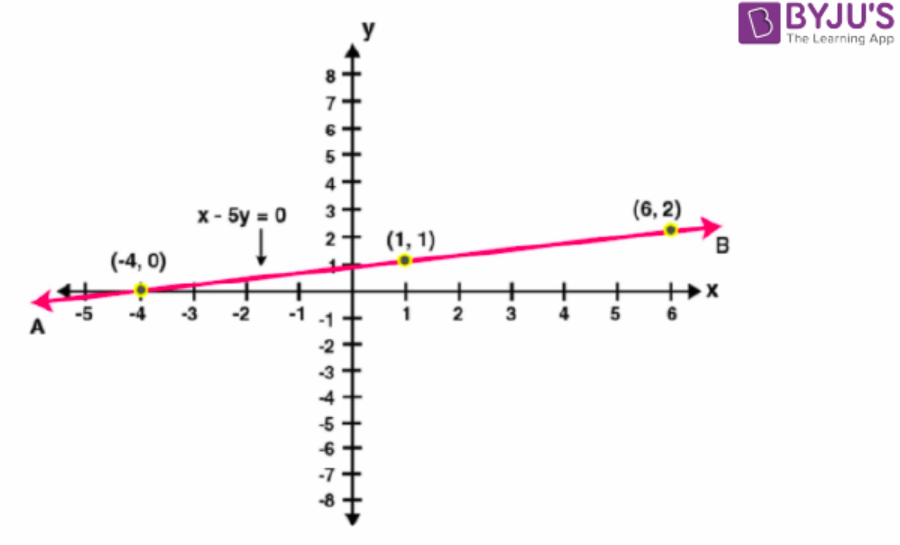(viii) Given 5x + y + 5 = 0

y = -5x – 5

If x = 0, then y = -5 (0) – 5 = 0 – 5 = -5

If x = -1, then y = -5 (-1) – 5 = 5 – 5 = 0

If x = -2, then y = -5 (-2) – 5 = 10 – 5 = 5

The points are

 x 0 -1 -2 y -5 0 5

Plotting these points, we get the required graph as given below: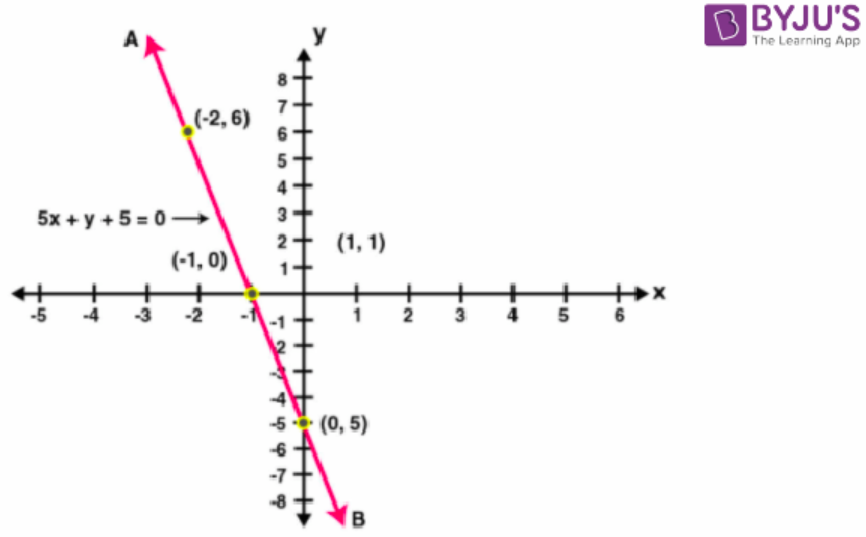2. Draw the graph for each equation given below; hence find the co-ordinates of the

points where the graph drawn meets the co-ordinate axes:

(i) 1/3 x + 1/5 y = 1

(ii) (2x + 15)/3 = y – 1

Solution:

(i) Given 1/3 x + 1/5 y = 1

Taking LCM. We get

5x + 3y / 15 = 1

On cross multiplication, we get

5x + 3y = 15

3y = 15 – 5x

y = (15 – 5x)/3

If x = 0, then y = (15 – 5 × 0)/3 = (15 – 0)/3 = 5

If x = 3, then y = (15 – 5 × 3)/3 = (15 – 15)/3 = 0

If x = -3, then y = (15 – 5 × -3)/3 = (15 + 15)/3 = 10

The points are

 X 0 3 -3 Y 5 0 10

Plotting these points, we get the required graph as given below: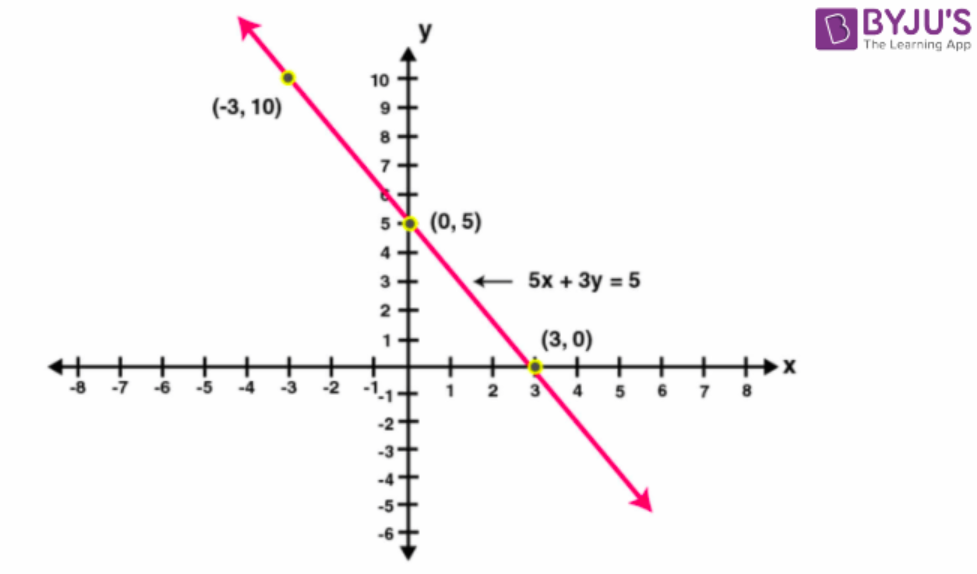Hence the graph meets the coordinate axis at (3, 0) and (0, 5)

(ii) Given (2x + 15)/3 = y – 1

On cross multiplication we get

2x + 15 = 3(y – 1)

2x + 15 = 3y – 3

2x – 3y = -15 – 3

2x – 3y = -18

-3y = -18 – 2x

y = (-18 – 2x)/-3

If x = 0, then y = (- 18 – 2 × 0)/ – 3 = (-18 – 0)/-3 = 6

If x = -3, then y = (- 18 – 2 × -3)/ – 3 = (-18 + 6)/-3 = 4

If x = -6, then y = (- 18 – 2 × -6)/ – 3 = (-18 + 12)/-3 = 2

The points are

 x 0 -3 -6 y 6 4 2

Plotting these points, we get the required graph as given below: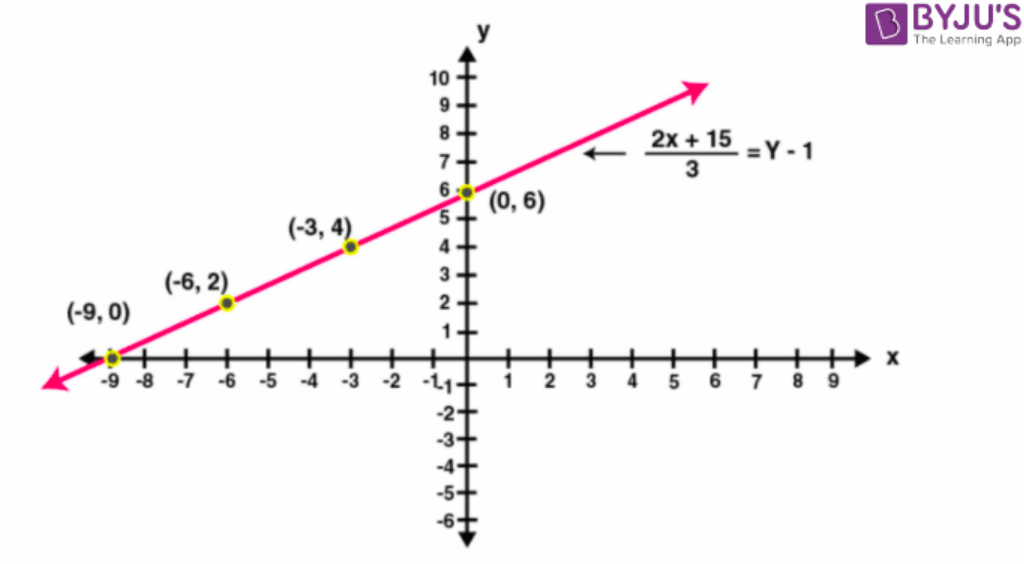Hence, the graph meets the coordinate axis at (-9, 0) and (0, 6)

3. Draw the graph of the straight line given by the equation 4x – 3y + 36 = 0. Calculate the area of the triangle formed by the line drawn and the co-ordinate axes.

Solution:

Given 4x – 3y + 36 = 0

4x – 3y = – 36

-3y = -36 – 4x

3y = 36 + 4x

y = (36 + 4x)/3

If x = -6, then y = (36 + 4 × -6)/ 3 = (36 – 24)/3 = 4

If x = -3, then y = (36 + 4 × -3)/ 3 = (36 – 12)/3 = 8

If x = -9, then y = (36 + 4 × -9)/ 3 = (36 – 36)/3 = 0

The points are

 X -9 -3 -6 Y 0 8 4

Plotting these points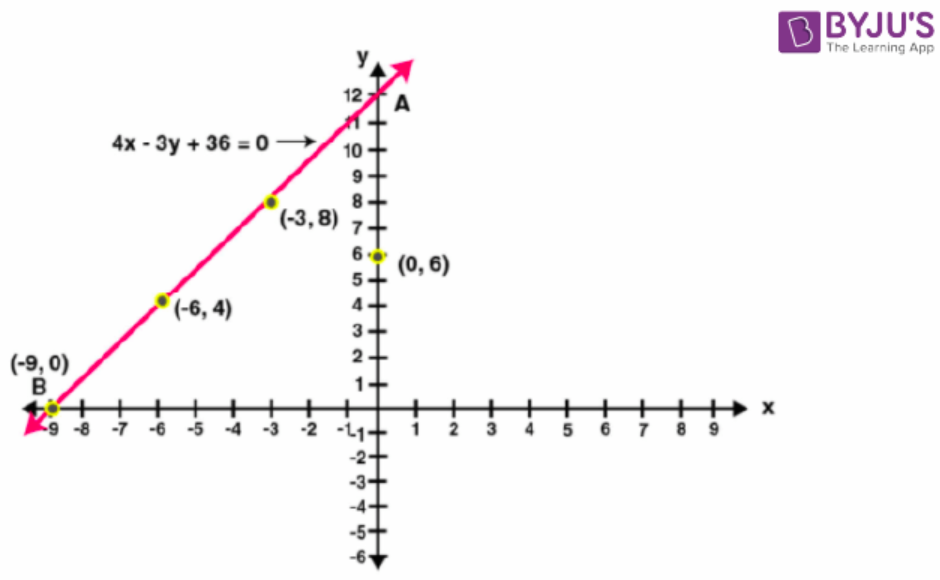Hence the straight line cuts the coordinate axis at A(0, 12) and B(-9, 0)

Therefore, the triangle AOB is formed

Area of triangle AOB = ½ × AO × OB

= ½ × 12 × 9

= 54 sq. units.

Area of triangle AOB = 54 sq. units.

4. Draw the graph of the equation 2x – 3y – 5 = 0. From the graph, find:

(i) x1, the value of x, when y = 7

(ii) x2, the value of x, when y = -5

Solution:

Given 2x – 3y – 5 = 0

2x = 3y + 5

x = (3y + 5)/2

If y = 1, then x = (3 × 1 + 5)/ 2 = 8/2 = 4

If y = 3, then x = (3 × 3 + 5)/ 2 = 9 + 5/2 = 14/2 = 7

If y = -1, then x = (3 × -1 + 5)/ 2 = 5 – 3/2 = 2/2 = 1

The points are

 X 4 7 1 Y 1 3 -1

Plotting these points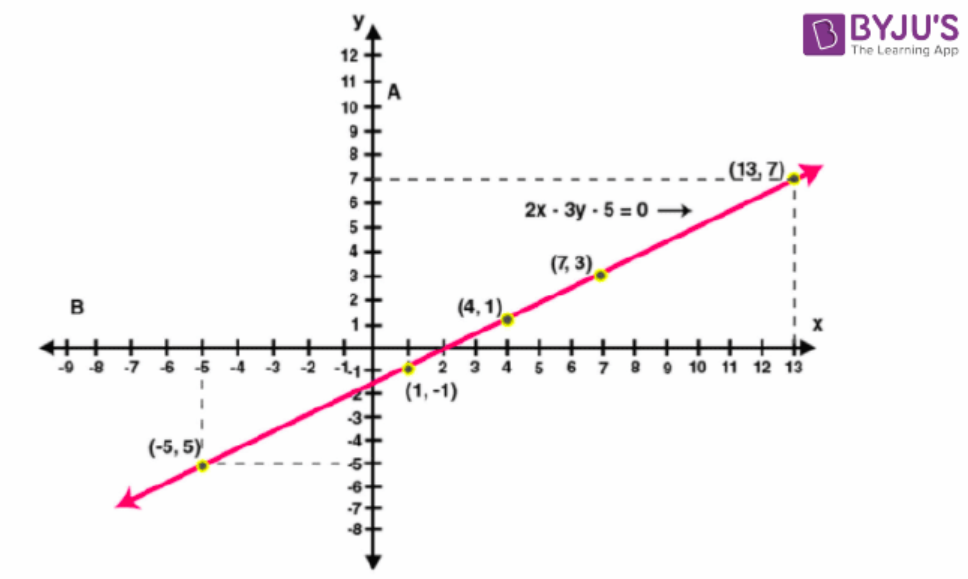The value of x, when y = 7

We have the equation of the line as

x = (3y + 5)/2

now substitute y = 7 and x = x1

x1 = [3 (7) + 5]/2 = (21 + 5)/2 = 26/2 = 13

the value of x when y = -5

now substitute y = -5 and x = x2

x2 = [3 (-5) + 5)/2 = (-15 + 5)/2 = -10/2 = -5

5. Draw the graph of the equation 4x + 3y + 6 = 0. From the graph, find:

(i) y1, the value of y, when x = 12

(ii) y2, the value of y, when x = -6

Solution:

Given 4x + 3y + 6 = 0

3y = -4x – 6

y = (-4x – 6)/3

If x = 0, then y = (-4 × 0 – 6)/ 3 = -6/3 = -2

If x = 3, then y = (-4 × 3 – 6)/ 3 = -12 – 6/3 = -6

If x = -3, then y = (-4 × -3 – 6)/ 3 = 12 – 6/3 = 2

The points are

 X 0 3 -3 Y -2 -6 2

Plotting these points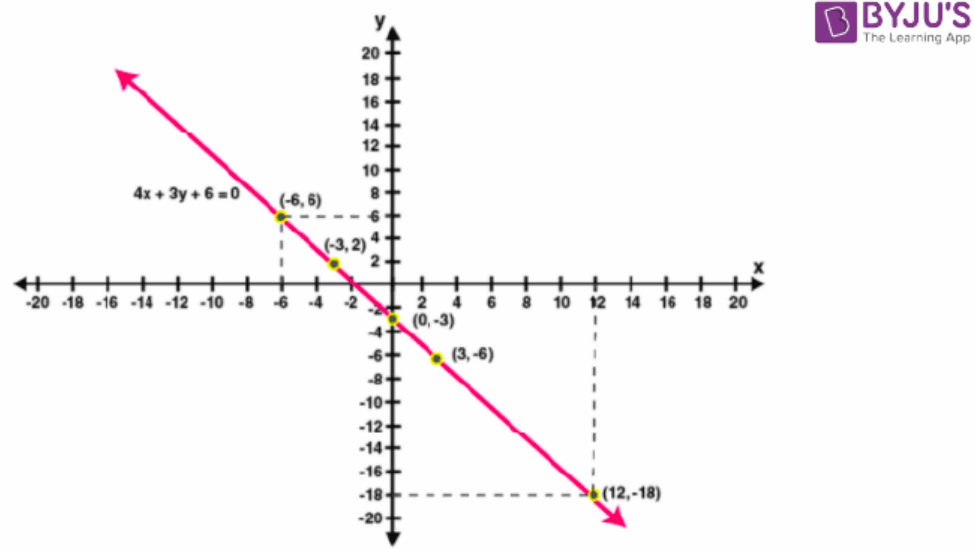The value of y, when x = 12

We have the equation of the line as

y = (-4x – 6)/3

now substitute x = 12 and y = y1

y1 = [- 4 (12) – 6]/3 = (-48 – 6)/3 = -54/3 = -18

the value of y, when x = -6

now substitute x = -6 and y = y2

y1 = [- 4 (-6) – 6]/3 = (24 – 6)/3 = 18/3 = 6

6. Use the table given below to draw the graph.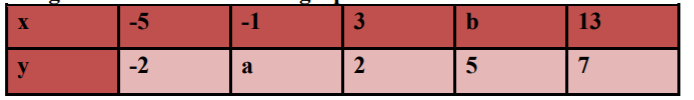From your graph, find the values of ‘a’ and ‘b’. State a linear relation between the variables x and y.

Solution: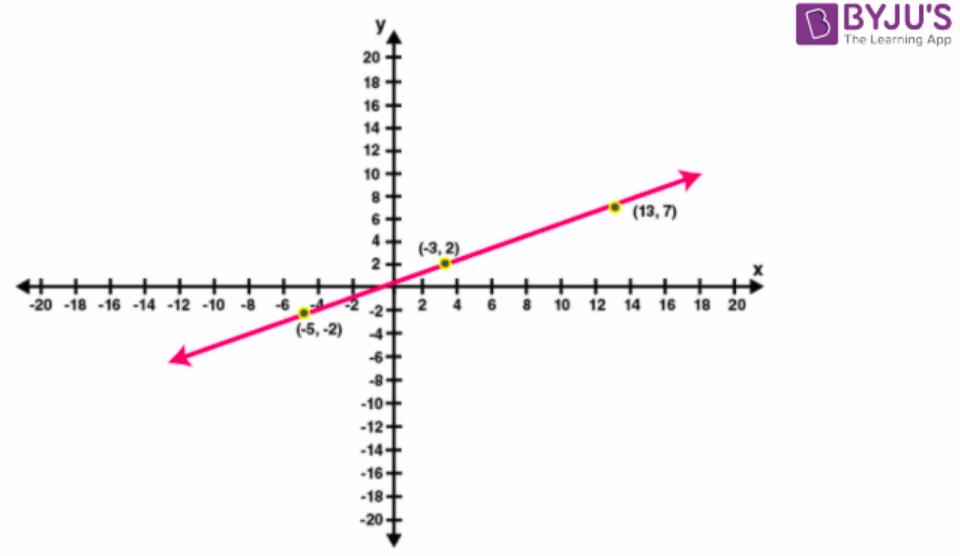When x = -1, y = 0 that is a = 0

When y = 5, x = 9 that is b = 9

Let y = px + q ………. (1)

Be a linear relation between x and y

Substitute x = 9 and y = 5 in the equation (1) we get

5 = 9p + q …….. (2)

Substitute x = -1 and y = 0 in the equation (1) we get

0 = -p + q …. (3)

Subtracting (3) from (2) we have

5 = 10p

p = 5/10

p = ½

from 3 we have

p = q

therefore, q = ½

thus linear relation is

y = ½ x + ½

y = (x + 1)/2

7. Draw the graph obtained from the table below: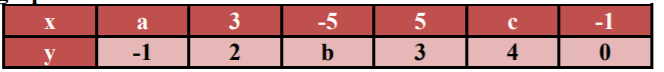Use the graph to find the values of a, b and c. State a linear relation between the variables x and y.

Solution: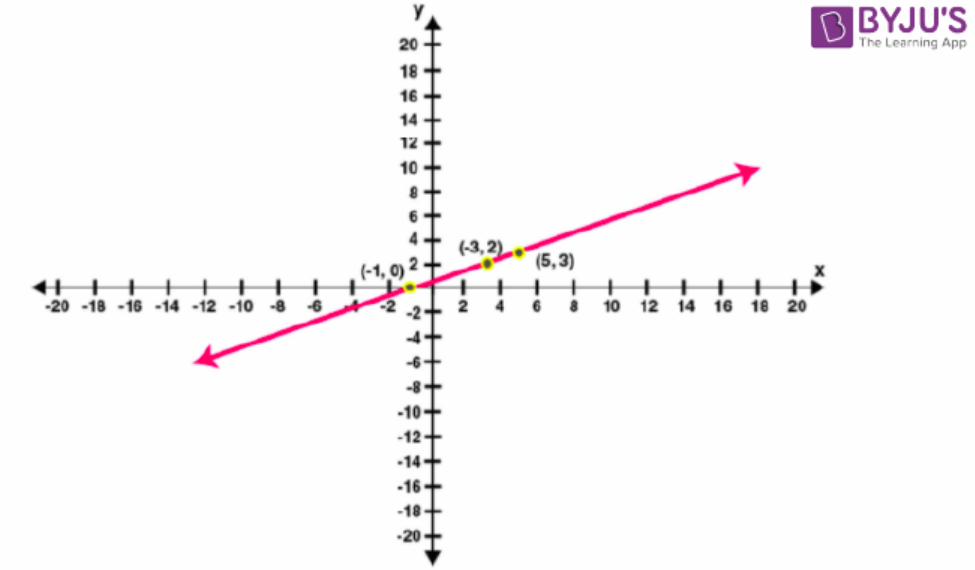When y = -1, then x = -3

a = -3

when x = -5, then y = -2

b = -2

when y = 4, then x = 7

c = 7

let y = px + q ……… (1)

be a linear relation between x and y

substitute x = -3 and y = -1 in the equation (1)

– 1 = -3p + q ………. (2)

substitute x = -5 and y = -2 in the equation (1)

-2 = -5p + q ……… (3)

Subtracting (3) from (2) we have

1 = 2p

p = ½

from (3), We have

-2 = -5p + q

-2 = -5 (½) + q

-4 = -5 + 2q

2q = 5 – 4

2q = 1

q = ½

thus, the linear relation is

y = px + q

y = ½ x + ½

y = (x + 1)/2

8. A straight line passes through the points (2, 4) and (5, -2). Taking 1 cm = 1 unit; mark these points on a graph paper and draw the straight line through these points. If points (m, -4) and (3, n) lie on the line drawn; find the values of m and n.

Solution:

The table is

 X 2 3 5 m Y 4 n -2 -4

Plotting these points as shown in the above table, we get the following graph: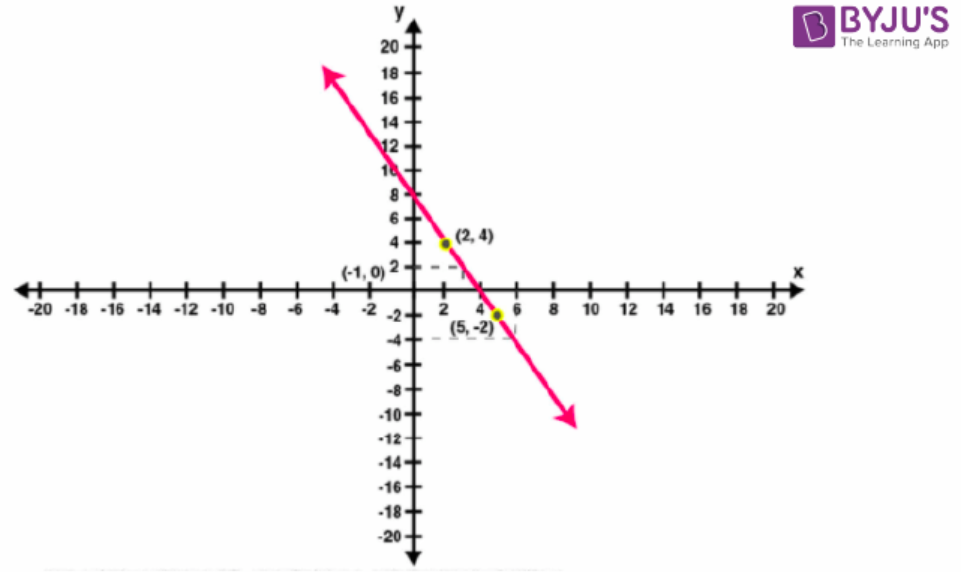Now draw a line x = 3, parallel to y – axis to meet the line

It meets the line at y = 2

Therefore n = 2

Now draw a line y = -4 parallel to x – axis to meet the line

It meets the line at x = 6

Therefore m = 6

Thus, the value of m and n are 6 and 2 respectively

9. Draw the graph (straight line) given by equation x – 3y = 18. If the straight-line drawn passes through the points (m, -5) and (6, n); find the values of m and n.

Solution:

According to the question

x – 3y = 18

– 3y = x – 18

y = (x – 18)/3

the points are

 x 9 0 6 3 Y -3 -6 -4 -5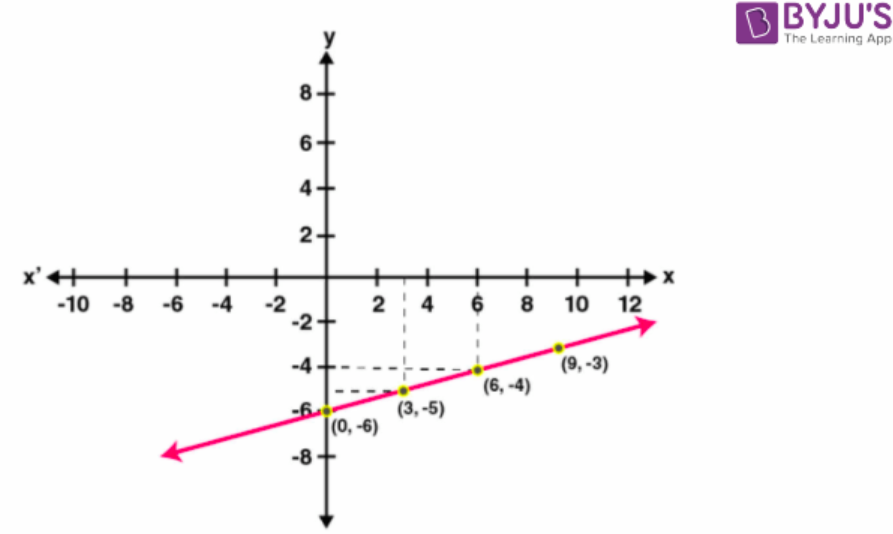Hence, from the graph we can conclude that M = 3 AND N = 4

10. Use the graphical method to find the value of k, if:

(i) (k, -3) lies on the straight line 2x + 3y = 1

(ii) (5, k – 2) lies on the straight line x – 2y + 1 = 0

Solution:

(i) Given 2x + 3y = 1

3y = 1 – 2x

y = ( 1 – 2x)/3

the points are

 x -1 2 5 Y 1 -1 -3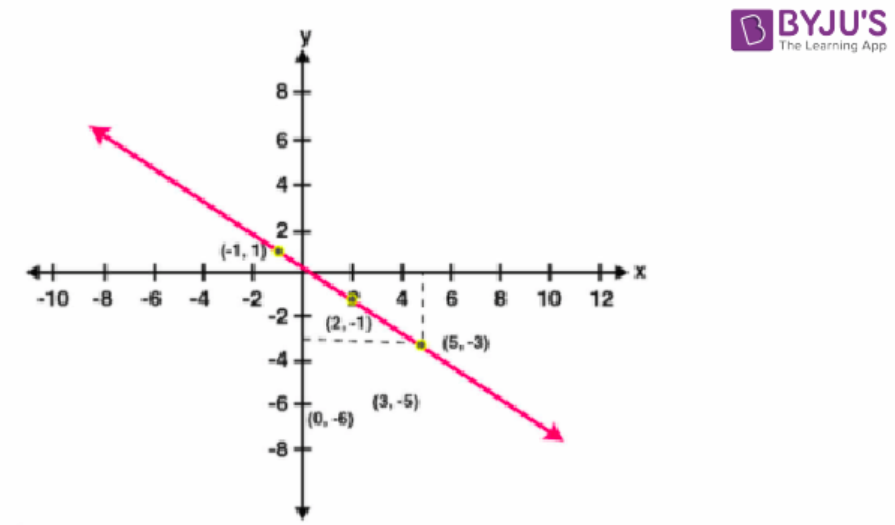Hence from the graph we can say k = 5

(ii) Given x – 2y + 1 = 0

2y = x + 1

y = (x + 1)/2

the points are

 x 1 3 5 Y 1 2 3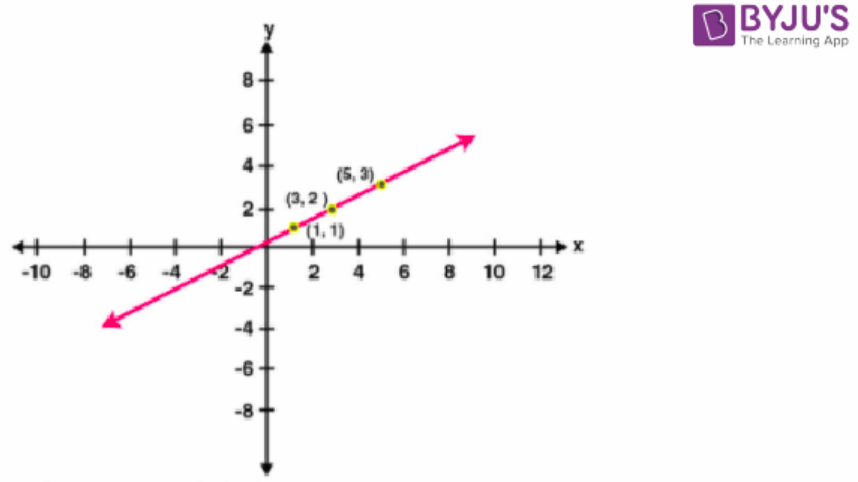Hence, from the graph we can say k – 2 = 3

Implies k = 5

Exercise 27b Page: 329

1. Solve, graphically, the following pairs of equations:

(i) x – 5 = 0

y + 4 = 0

Solution:

(i) Given x – 5 = 0

y + 4 = 0

given equations can be written as

x – 5 = 0

x = 5

y + 4 = 0

y = -4

the graph of following equations given below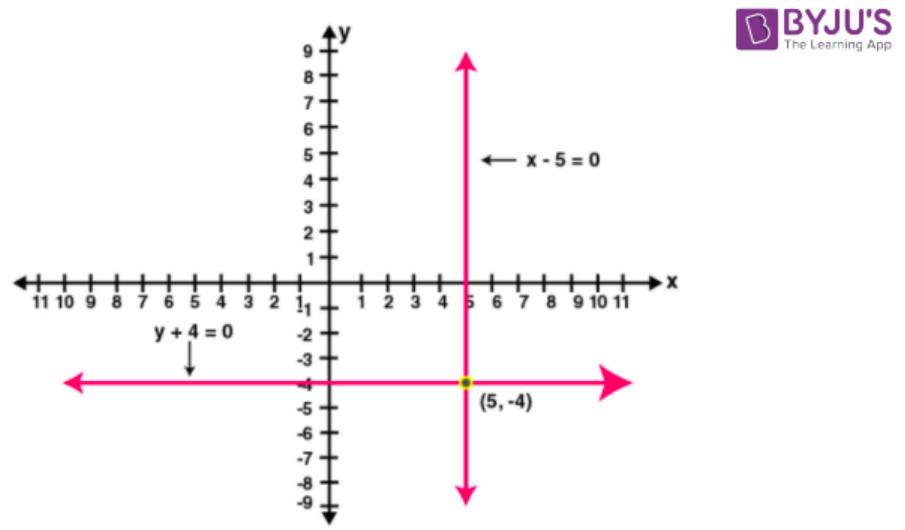(ii) 2x + y = 23

4x – y = 19

Solution:

Given 2x + y = 23

y = 23 – 2x

the points table for y = 23 – 2x

 X 5 10 15 Y 13 3 -7

Also we have

4x – y = 19

y = 4x – 19

the points table for y = 4x – 19

 X 3 4 6 Y -7 -3 5

Plotting these points, we get the required graph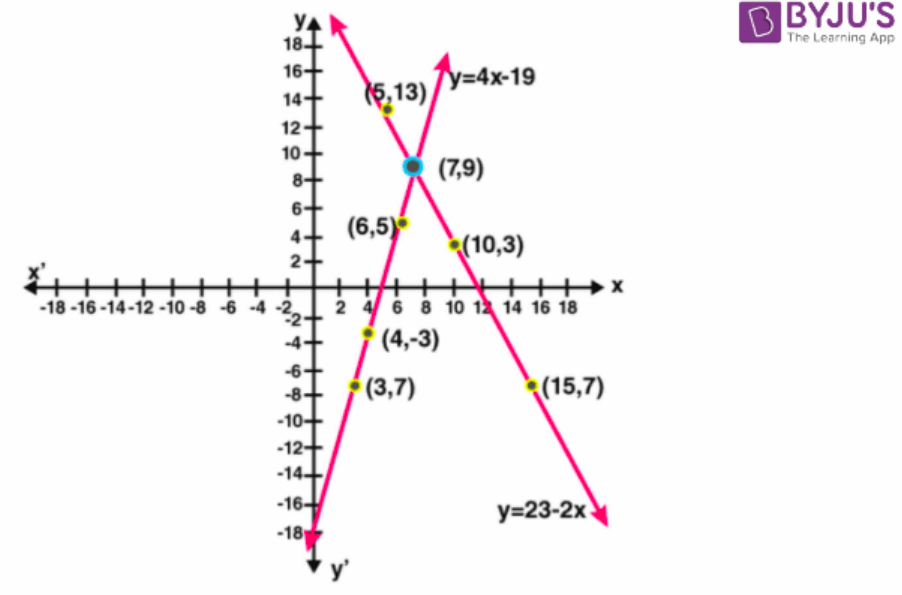By seeing the graph, we can conclude that given two lines intersect at (7, 9)

(iii) 3x + 7y = 27

8 – y = 5/2 x

Solution:

Given 3x + 7y = 27

3x = 27 – 7y

x = (27 – 7y)/3

the table for 3x + 7y = 27 is

 x 9 2 -5 y 0 3 6

Also we have

8 – y = 5/2 x

x = (8 – y)2/5

the table for 5x + 2y = 16 is

 x 2 4 0 y 3 -2 8

Plotting the points, we get the following required graph: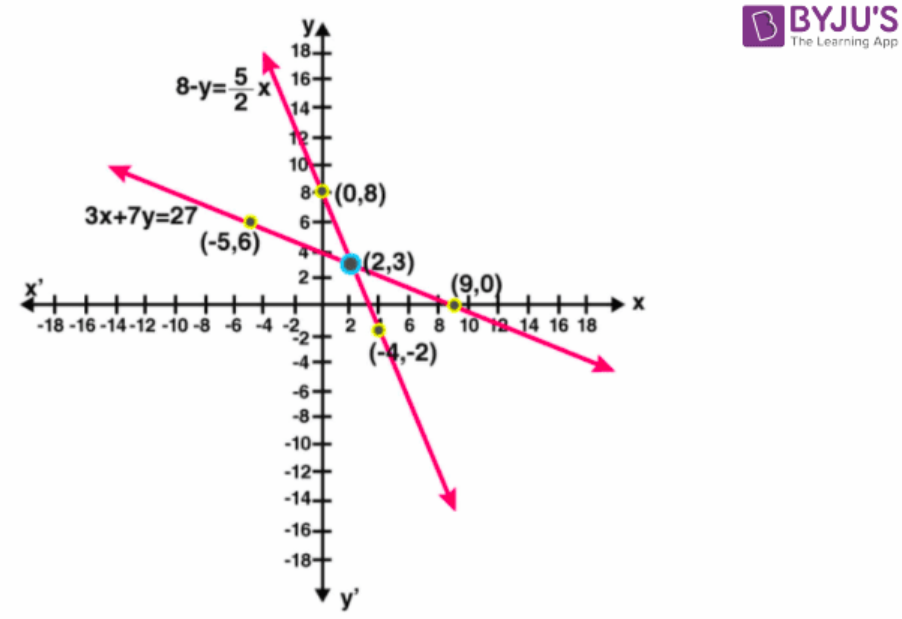Intersection point = (2, 3)

(iv) (x + 1)/4 = 2/3 (1 – 2y)

(2 + 5y)/3 = x/7 – 2

Solution:

(x + 1)/4 = 2/3 (1 – 2y)

(x + 1)/4 = 2/3 – 4y/3

Multiply above equation on both sides we get

12
× (x + 1)/4 = 12 × 2/3 – 12 × (4y/3)

3 (x + 1) = 8 – 16y

3x + 3 = 8 – 16y

3x – 5 = – 16y

x = (5 – 16y)/3

the table for (x + 1)/4 = 2/3 )1 – 2y) is

 X 7 -9 23 Y -1 2 -4

Also we have

(2 + 5y)/3 = x/7 – 2

Multiply both sides by 21 we get

21 × (2 + 5y)/3 = 21 × x/7 – 21 × 2

7(2 + 5y) = 3x – 42

14 + 35y = 3x – 42

3x = 14 + 35 y + 42

3x = 56 + 35y

X = (56 + 53y)/3

The table for (2 + 5y)/3 = x/7 – 2

 X 7 -28 42 Y -1 -4 2

Plotting the points, we get the following required graph: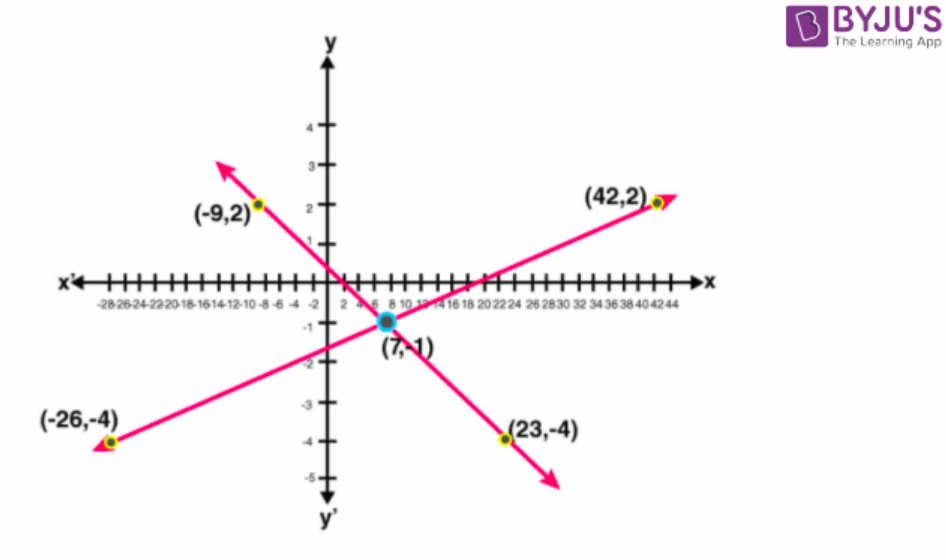Intersection point = (7, -1)

2. Solve graphically the simultaneous equations given below. Take the scale as 2 cm = 1 unit

on both the axes.

x – 2y – 4 = 0

2x + y = 3

Solution:

Given x – 2y – 4 = 0

2x + y = 3

The table for x – 2y – 4 = 0 is

 X 4 6 2 Y 0 1 -1

Also we have

2x + y = 3

2x = 3 – y

x = (3 – y)/2

the table for 2x + y = 3 is

 X 1 0 2 Y 1 3 -1

Plotting the above points we get the following graph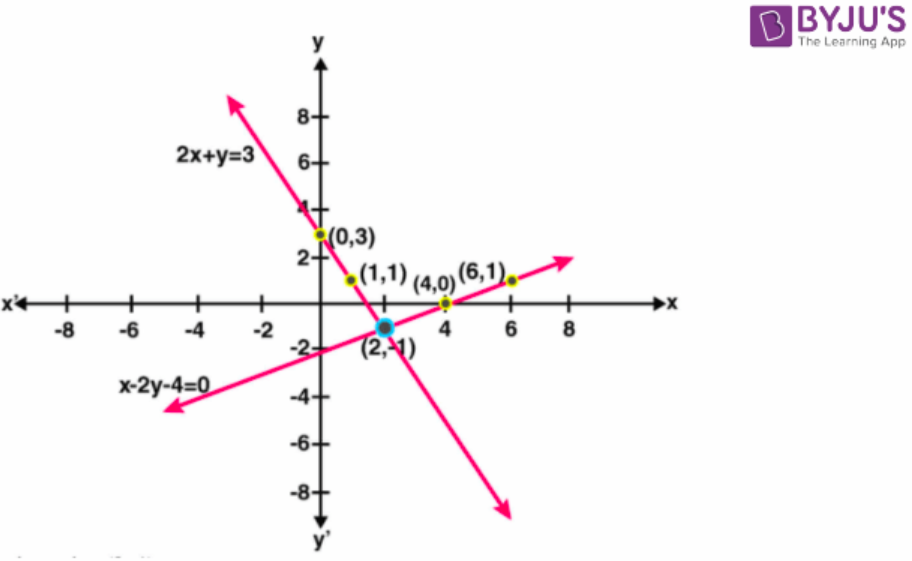Intersection point = (2, -1)

3. Use graph paper for this question. Draw the graph of 2x – y – 1 = 0 and 2x + y = 9 on the same axes. Use 2 cm = 1 unit on both axes and plot only 3 points per line. Write down the co-ordinates of the point of intersection of the two lines.

Solution:

Given 2x – y – 1 = 0

2x = y + 1

X = (y + 1)/2

The table for 2x – y – 1 = 0 is

 X 2 1 0 Y 3 1 -1

Also we have

2x + y = 9

2x = 9 – y

x = (9 – y)/2

the table for 2x + y = 9 is

 X 4 3 5 Y 1 3 -1

Plotting the above points we get,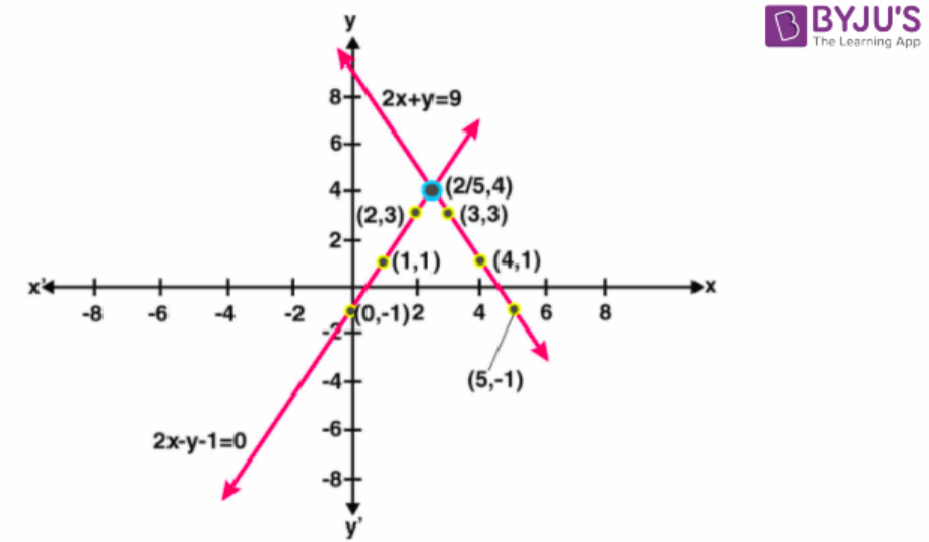Intersection point = (2.5, 4)

4. Use graph paper for this question. Take 2 cm = 2 units on x-axis and 2 cm = 1 unit on y-axis.

Solve graphically the following equations:

3x + 5y = 12; 3x – 5y + 18 = 0

(Plot only three points per line)

Solution:

Given 3x + 5y = 12

3x = 12 – 5y

x = (12 – 5y)/3

the table for 3x + 5y = 12 is

 X 4 -1 -6 Y 0 3 -1

Also we have

3x – 5y + 18 = 0

3x = 5y – 18

x = (5y – 18)/3

the table for 3x – 5y + 18 = 0 is

 X -6 4 -1 Y 0 6 3

Plotting the above points we get required graph: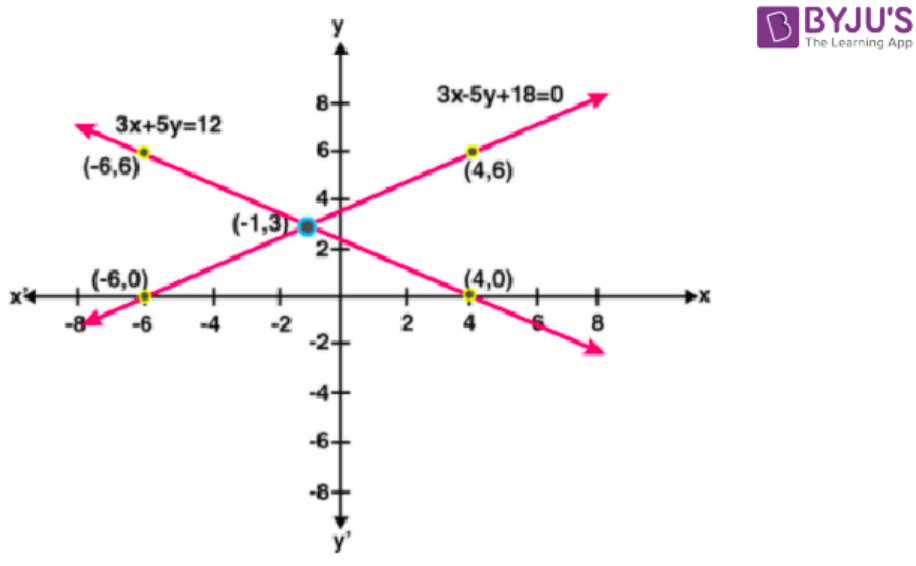Intersection point (-1, 3)

5. Use graph paper for this question. Take 2 cm = 1 unit on both the axes.

(i) Draw the graphs of x + y + 3 = 0 and 3x – 2y + 4 = O. Plot only three points per line.

(ii) Write down the co-ordinates of the point of intersection of the lines.

(iii) Measure and record the distance of the point of intersection of the lines from the origin in cm.

Solution:

(i) Given x + y + 3 = 0

x = -3 – y

the table for x + y + 3 = 0 is

 X 1 0 -2 Y -4 -3 -1

Also we have

3x – 2y + 4 = 0

3x = 2y – 4

x = (2y – 4)/3

the table for 3x – 2y + 4 = 0 is

 X 0 -2 -2/3 Y 2 -1 1

Plotting the above points we get the requires graph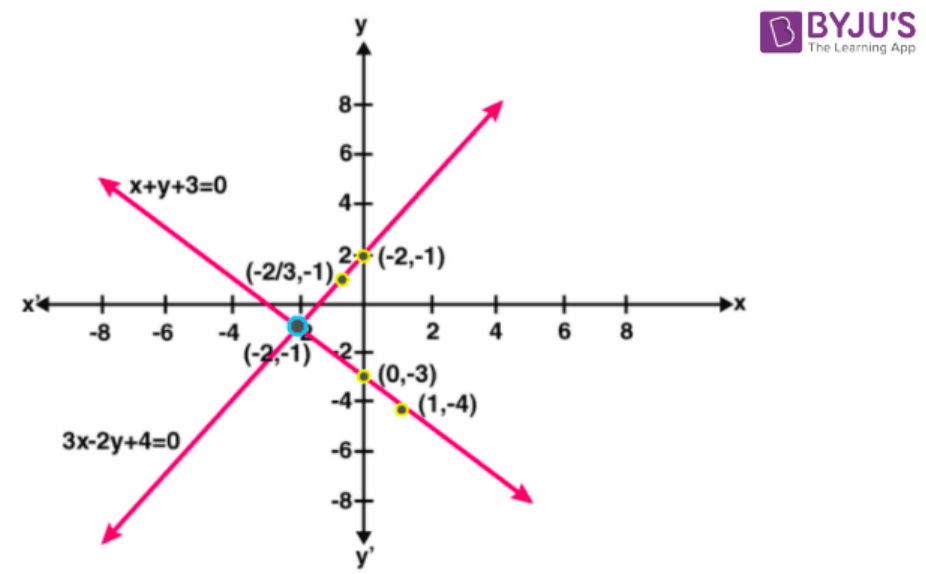(ii) intersection points = (-2, -1)

(iii) Now applying Pythagoras theorem,

The distance from the origin = √[(-2 – 0)2 + (-1 = 0)2]

= √(22 + 12)

= √(4 + 1)

= √5

= 2. 2 cm (approximately)

6. The sides of a triangle are given by the equations y – 2 = 0; y + 1 = 3 (x – 2) and x + 2y = O.

Find, graphically:

(i) the area of triangle;

(ii) the co-ordinates of the vertices of the triangle.

Solution:

Given y – 2 = 0

y = 2

y + 1 = 3 (x – 2)

y = 3x – 6 – 1

y = 3x – 7

the table for y + 1 = 3(x – 2) is

 X 1 2 3 Y -4 -1 2

Also we have

x + 2y = 0

x = -2y

the table for x + 2y = 0 is

 X -4 4 -6 Y 2 -2 3

Plotting the above points we get the required graph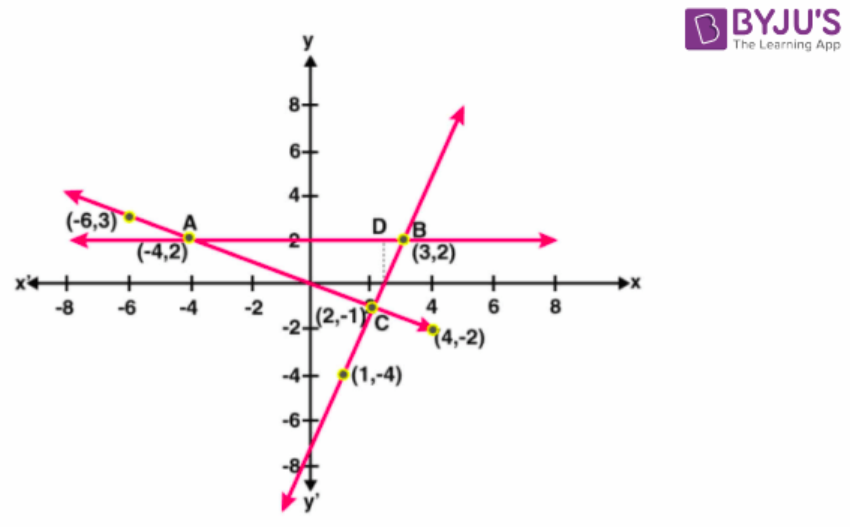The area of triangle ABC = ½
× AB
× CD

= ½ × 7 × 3

= 21/2

= 10. 5 sq. units

(ii) The coordinates of the vertices of the triangle are (-4, 2), (3. 2) and (2, -1)

7. By drawing a graph for each of the equations 3x + y + 5 = 0; 3y – x = 5 and 2x + 5y = 1 on the same graph paper; show that the lines given by these equations are concurrent (i.e. they pass through the same point). Take 2 cm = 1 unit on both the axes.

Solution:

Given 3x + y + 5 = 0

y = -3x – 5

the table of 3x + y + 5 = 0 is

 X 1 -3 -2 Y -8 4 1

3y – x = 5

x = 3y – 5

the table of 3y – x = 5 is

 X -2 1 7 Y 1 2 4

2x + 5y = 1

2x = 1 – 5y

x = (1 – 5y)/2

the table of 2x + 5y = 1 is

 X 3 -7 -2 Y -1 3 1

Plotting the above points, we get the required graph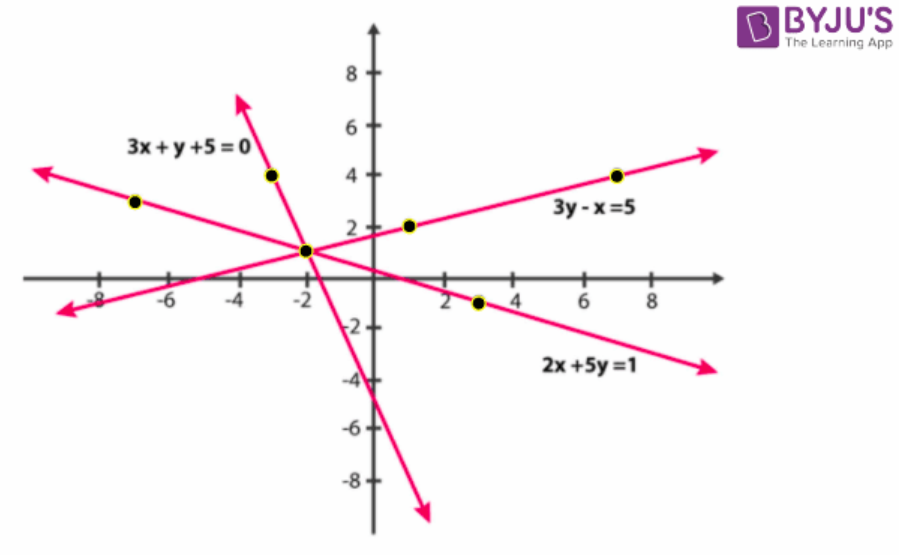Hence,

According to the graph the lines of the graphs are concurrent.

8. Using a scale of 1 cm to 1 unit for both the axes, draw the graphs of the following equations: 6y = 5x + 10, y = 5x – 15.

From the graph find:

(i) the co-ordinates of the point where the two lines intersect;

(ii) the area of the triangle between the lines and the x-axis.

Solution:

Given 6y = 5x + 10

y = (5x + 10)/6

the table for 6y = 5x + 10 is

 X 4 -2 -8 Y 5 0 -5

Also we have

Y = 5x – 15

The table of y = 5x – 15 is

 X 3 4 5 Y 0 5 10

Plotting the given points we get the required graph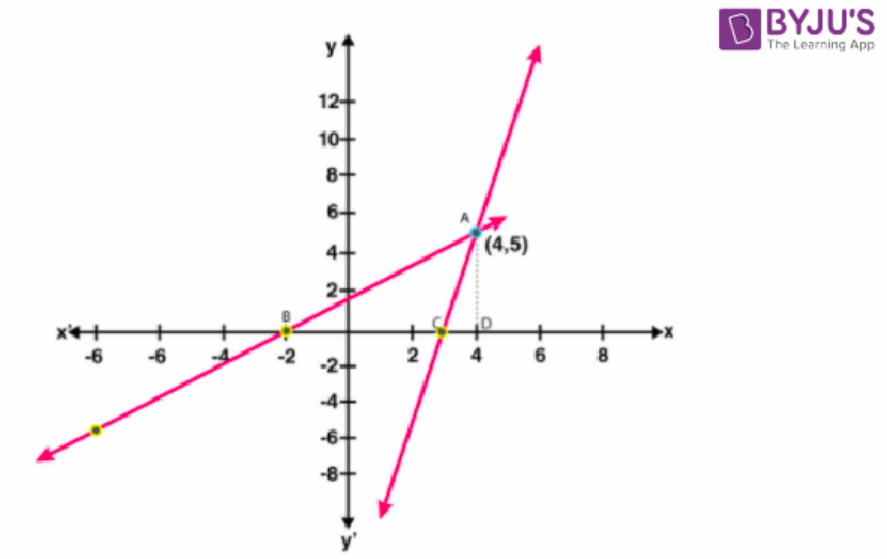(i) The two lines intersect at (4. 5)

And BC = 5 units

(ii) The area of triangle = ½ × BC × AD

= ½ × 5 × 5

= 25/2

= 12.5 Sq. units

9. The cost of manufacturing x articles is Rs.(50 + 3x). The selling price of x articles is Rs.4x. On a graph sheet, with the same axes, and taking suitable scales draw two graphs, first for the cost of manufacturing against no. of articles and the second for the selling price against number of articles.

(i) No. of articles to be manufactured and sold to break even (no profit and no loss),

(ii) The profit or loss made when

(a) 30

(b) 60 articles are manufactured and sold

Solution:

Given that CP is 50 + x

Table of CP

 x 0 10 20 30 40 50 60 CP 50 80 110 140 170 200 230

And SP = 4x

The table of SP

 x 0 10 20 30 40 50 60 SP 0 40 80 120 160 200 240

Now plotting these points on the graph we get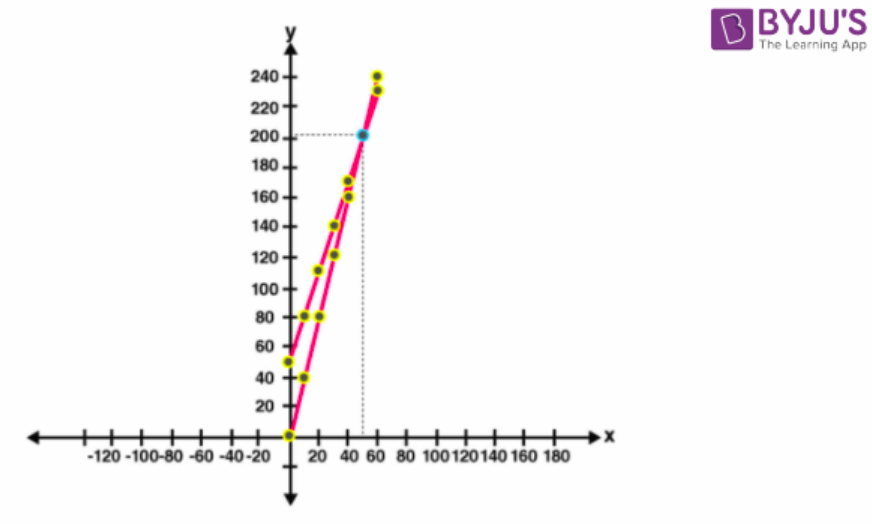(i) No. of articles to be manufactured and sold are 50 when there is no loss and no profit.

C.P = S.P = Rs.200

(ii)(a) On article 30,

C.P = Rs.140 and S.P. = 120

Therefore Loss = 140 – 120 = Rs.20

(b) On article 60,

C.P.=Rs.230 and S.P.= Rs.240

Therefore Profit = 240 – 230 = Rs.10

10. Find graphically, the vertices of the triangle whose sides have the equations 2y – x = 8; 5y – x = 14 and y – 2x = 1 respectively. Take 1 cm = 1 unit on both the axes.

Solution:

Given 2y – x = 8

y = (8 + x)/2

the table of 2y – x = 8 is

 X -6 -2 0 Y 1 3 4

5y – x = 14

x = 5y – 14

the table of x = 5y – 14 is

 X -9 -4 1 Y 1 2 3

y – 2x = 1

y = 1 + 2x

the table of y – 2x = 1 is

 X 2 -2 0 Y 5 -3 1

Now plotting these points we get required graph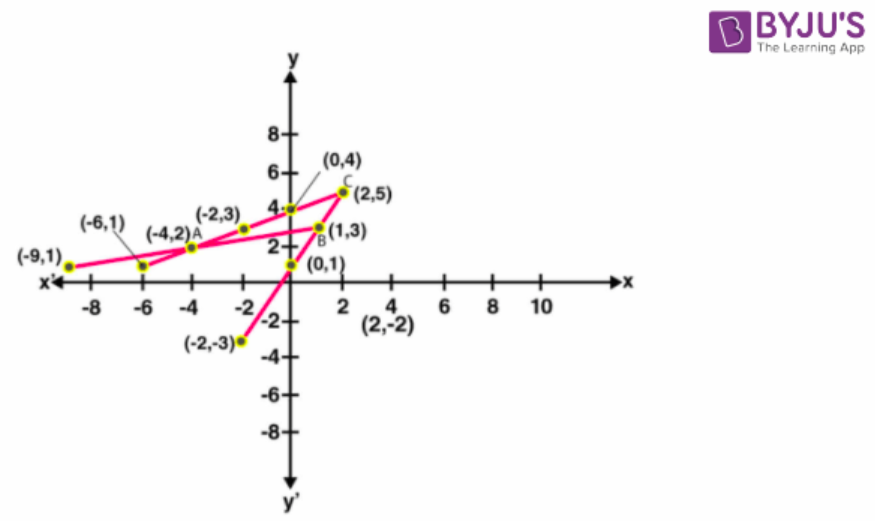The coordinates of the vertices of the triangle = A (-4, 2), B (1, 3) and C (2, 5)

## Selina Solutions for Class 9 Maths Chapter 27- Graphical Solution

The Chapter 27, Graphical Solution, is composed of 2 exercises and the solutions given here contain answers for all the questions present in these exercises. Let us have a look at some of the topics that are being discussed in this chapter.

27.1 Graphs of Linear Equations in Two Variables

27.2 Solution of Simultaneous Linear Equations, Graphically

## Selina Solutions for Class 9 Maths Chapter 27- Graphical Solution

The solution obtained from the intersecting point of the lines is known as the Graphical Solution. In this chapter, students are taught the method of solving the problems related to Graphical Solution. Read and learn the Chapter 27 of Selina textbook to familiarize with the concepts related to Graphical Solution. Learn the Selina Solutions for Class 9 effectively to score high in the examination.